# JP5854695B2 - Robot apparatus control method and robot apparatus - Google Patents

## Info

Publication number
JP5854695B2
JP5854695B2 JP2011175521A JP2011175521A JP5854695B2 JP 5854695 B2 JP5854695 B2 JP 5854695B2 JP 2011175521 A JP2011175521 A JP 2011175521A JP 2011175521 A JP2011175521 A JP 2011175521A JP 5854695 B2 JP5854695 B2 JP 5854695B2
Authority
JP
Japan
Prior art keywords
command value
value
driving force
torque command
Prior art date
Legal status (The legal status is an assumption and is not a legal conclusion. Google has not performed a legal analysis and makes no representation as to the accuracy of the status listed.)
Active
Application number
JP2011175521A
Other languages
Japanese (ja)
Other versions
JP2012086354A (en
Inventor

Original Assignee
キヤノン株式会社
Priority date (The priority date is an assumption and is not a legal conclusion. Google has not performed a legal analysis and makes no representation as to the accuracy of the date listed.)
Filing date
Publication date
Priority to JP2010212314 priority Critical
Priority to JP2010212314 priority
Application filed by キヤノン株式会社 filed Critical キヤノン株式会社
Priority to JP2011175521A priority patent/JP5854695B2/en
Priority claimed from US13/226,784 external-priority patent/US8812161B2/en
Publication of JP2012086354A publication Critical patent/JP2012086354A/en
Publication of JP5854695B2 publication Critical patent/JP5854695B2/en
Application granted granted Critical
Application status is Active legal-status Critical
Anticipated expiration legal-status Critical

## Images

•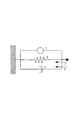•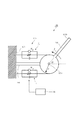•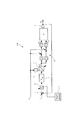•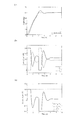•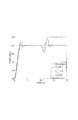•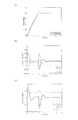•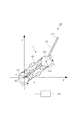•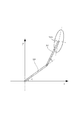•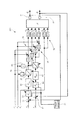•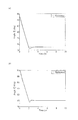•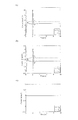•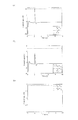•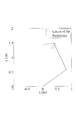•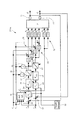•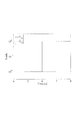•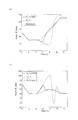•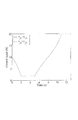•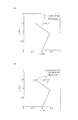•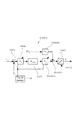•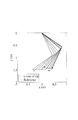•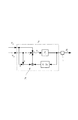•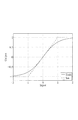•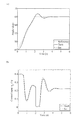•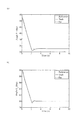•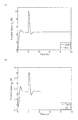•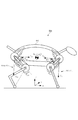•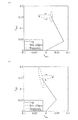•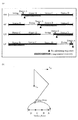•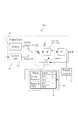••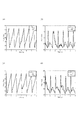•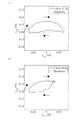•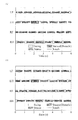•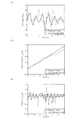•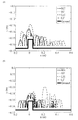•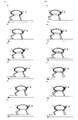•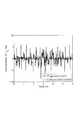## Classifications

• BPERFORMING OPERATIONS; TRANSPORTING
• B25HAND TOOLS; PORTABLE POWER-DRIVEN TOOLS; MANIPULATORS
• B25JMANIPULATORS; CHAMBERS PROVIDED WITH MANIPULATION DEVICES
• B25J9/00Programme-controlled manipulators
• B25J9/16Programme controls
• B25J9/1628Programme controls characterised by the control loop
• B25J9/1633Programme controls characterised by the control loop compliant, force, torque control, e.g. combined with position control
• BPERFORMING OPERATIONS; TRANSPORTING
• B25HAND TOOLS; PORTABLE POWER-DRIVEN TOOLS; MANIPULATORS
• B25JMANIPULATORS; CHAMBERS PROVIDED WITH MANIPULATION DEVICES
• B25J9/00Programme-controlled manipulators
• B25J9/10Programme-controlled manipulators characterised by positioning means for manipulator elements
• B25J9/1075Programme-controlled manipulators characterised by positioning means for manipulator elements with muscles or tendons
• BPERFORMING OPERATIONS; TRANSPORTING
• B25HAND TOOLS; PORTABLE POWER-DRIVEN TOOLS; MANIPULATORS
• B25JMANIPULATORS; CHAMBERS PROVIDED WITH MANIPULATION DEVICES
• B25J9/00Programme-controlled manipulators
• B25J9/16Programme controls
• B25J9/1628Programme controls characterised by the control loop
• B25J9/1641Programme controls characterised by the control loop compensation for backlash, friction, compliance, elasticity in the joints
• GPHYSICS
• G05CONTROLLING; REGULATING
• G05BCONTROL OR REGULATING SYSTEMS IN GENERAL; FUNCTIONAL ELEMENTS OF SUCH SYSTEMS; MONITORING OR TESTING ARRANGEMENTS FOR SUCH SYSTEMS OR ELEMENTS
• G05B2219/00Program-control systems
• G05B2219/30Nc systems
• G05B2219/39Robotics, robotics to robotics hand
• G05B2219/39201Control of joint stiffness
• GPHYSICS
• G05CONTROLLING; REGULATING
• G05BCONTROL OR REGULATING SYSTEMS IN GENERAL; FUNCTIONAL ELEMENTS OF SUCH SYSTEMS; MONITORING OR TESTING ARRANGEMENTS FOR SUCH SYSTEMS OR ELEMENTS
• G05B2219/00Program-control systems
• G05B2219/30Nc systems
• G05B2219/39Robotics, robotics to robotics hand
• G05B2219/39452Select with mouse button a coordinate plane for micromanipulation
• GPHYSICS
• G05CONTROLLING; REGULATING
• G05BCONTROL OR REGULATING SYSTEMS IN GENERAL; FUNCTIONAL ELEMENTS OF SUCH SYSTEMS; MONITORING OR TESTING ARRANGEMENTS FOR SUCH SYSTEMS OR ELEMENTS
• G05B2219/00Program-control systems
• G05B2219/30Nc systems
• G05B2219/39Robotics, robotics to robotics hand
• G05B2219/39454Rubber actuator, two muscle drive, one for extension other for traction
• YGENERAL TAGGING OF NEW TECHNOLOGICAL DEVELOPMENTS; GENERAL TAGGING OF CROSS-SECTIONAL TECHNOLOGIES SPANNING OVER SEVERAL SECTIONS OF THE IPC; TECHNICAL SUBJECTS COVERED BY FORMER USPC CROSS-REFERENCE ART COLLECTIONS [XRACs] AND DIGESTS
• Y10TECHNICAL SUBJECTS COVERED BY FORMER USPC
• Y10STECHNICAL SUBJECTS COVERED BY FORMER USPC CROSS-REFERENCE ART COLLECTIONS [XRACs] AND DIGESTS
• Y10S901/00Robots
• Y10S901/01Mobile robot
• YGENERAL TAGGING OF NEW TECHNOLOGICAL DEVELOPMENTS; GENERAL TAGGING OF CROSS-SECTIONAL TECHNOLOGIES SPANNING OVER SEVERAL SECTIONS OF THE IPC; TECHNICAL SUBJECTS COVERED BY FORMER USPC CROSS-REFERENCE ART COLLECTIONS [XRACs] AND DIGESTS
• Y10TECHNICAL SUBJECTS COVERED BY FORMER USPC
• Y10STECHNICAL SUBJECTS COVERED BY FORMER USPC CROSS-REFERENCE ART COLLECTIONS [XRACs] AND DIGESTS
• Y10S901/00Robots
• Y10S901/02Arm motion controller
• Y10S901/09Closed loop, sensor feedback controls arm movement

## Description

The present invention relates to a control method of a robot apparatus that performs link angle control and rigidity control, and a robot apparatus. Preferably, it is applied to a work robot or a legged mobile robot.

In a manipulator control method, it has become important that an end effector (for example, a hand) provided at the end of a link can flexibly contact an object. If this is applied to an industrial robot, collaborative work between the robot apparatus and a human can be realized, and by fitting the direction of the flexibility of the hand, a part fitting work or the like is facilitated. Moreover, if this is applied to a legged mobile robot, it is possible to reduce the impact on the trunk by making a soft contact with the ground, and it is possible to stably walk on rough terrain by absorbing the steps.

In order to control the flexibility of the hand, impedance control for attaching a force sensor to the hand, control using an artificial muscle actuator, and the like are performed. It is known that the human muscle is not only an actuator but also a viscoelastic variable control mechanism. Among artificial muscles, pneumatic rubber artificial muscles represented by McKibben type artificial muscles are similar in muscle to viscoelastic properties. Then, by controlling the softness of the artificial muscle actuator arranged in the manipulator, the object can be contacted with the flexibility of any hand. However, artificial muscle actuators have difficulty in controllability because they have non-linear viscoelastic characteristics and need to be antagonistically arranged to generate force only in the contraction direction. It is known that there is.

On the other hand, a technology is disclosed in which a rubber artificial muscle actuator to which a compressed fluid is supplied is antagonistically arranged on a link, joint angle detection is performed, and feedback control is performed to control the swing angle of the link at the joint ( Patent Document 1).

Further, a technique for generating a feedforward input for simultaneously controlling a joint angle and flexibility of a hand using a manipulator model including a viscoelastic property of a muscle and a correction value calculation unit is disclosed (Patent Document 2). reference). In Patent Document 2, a joint angle when a control input is given using a model and the viscoelastic coefficient of the artificial muscle actuator are output and compared with a target value. Then, the error from the target value is propagated back to the correction value calculation unit, and the feedforward input is corrected. The operation of giving the corrected feedforward input to the model again is repeated, and the feedforward input is gradually obtained.

JP-A-63-68382 Japanese Patent No. 3436320

However, in Patent Document 1, feedback control is performed so that the swing angle of the link is detected and the target swing angle is obtained. However, only the swing angle of the link at the joint is controlled, and the link at the joint is controlled. Control that fluctuates the flexibility (rigidity) is not realized.

Furthermore, in Patent Document 2, the link swing angle and the link flexibility at the joint are simultaneously controlled by feedforward control. Since the feedforward control input is obtained by repeatedly correcting an error from the target value, the amount of calculation required for generating the feedforward control input is very large. Therefore, it is necessary to repeat the calculation every time the swing angle of the link or the target value of the flexibility of the joint connecting the link is changed, and when the target value is changed, the calculation takes time. There's a problem.

These problems are not limited to the case where an artificial muscle actuator is used as a drive unit for driving a link, but the same applies to a case where control is performed using a rotary motor as a drive unit.

Therefore, an object of the present invention is to provide a robot apparatus control method and a robot apparatus that perform link angle control and joint stiffness control by feedback control.

A control method for a robot apparatus according to the present invention includes a link mechanism having a link whose base end is swingably supported by a fixed member, and a drive unit that swings the link with respect to the fixed member, A first driving force command value indicating a driving force for swinging the link in the first swinging direction, and a drive for swinging the link in a second swinging direction opposite to the first swinging direction. Based on the difference with the second driving force command value indicating the force, the drive unit is operated to control the swing operation of the link, and the first driving force command value and the second driving force command value are controlled. In the control method of the robot apparatus that controls the rigidity of the joint between the fixed member and the link by operating the drive unit based on the sum of the distance between the link swing angle and the target swing angle with respect to the fixed member. A deviation calculating step for calculating the deviation, and compensating for the deviation; A torque command generation step of generating a torque command value T 1 to the torque command value T 1 of the absolute values, the length r and the first and second moment arm about the swing center point of the link A torque command correction step for correcting the torque command value T 1 in accordance with a constraint function that restricts a product value U 1 × r or less to a stiffness command value U 1 indicating the sum of the driving force command values, and the torque command correction step Using the torque command value T 1 corrected by the above, each driving force command value is determined by the following formula: (U 1 + T 1 / r) / 2, (U 1 −T 1 / r) / 2 A command value calculating step.

Further, the robot apparatus of the present invention includes a link having a base end swingably supported by a fixed member, a link mechanism having a drive unit that swings the link with respect to the fixed member, and the link as a first member. A first driving force command value indicating a driving force for swinging in a swinging direction, and a second driving force for swinging the link in a second swinging direction opposite to the first swinging direction. Based on the difference between the first driving force command value and the second driving force command value, the drive unit is operated based on the difference between the first driving force command value and the second driving force command value. And a control device that controls the rigidity of the joint between the fixed member and the link by operating the drive unit, and the control device detects a swing angle of the link with respect to the fixed member. The sensor calculates the deviation between the swing angle and the target swing angle. A deviation calculating section, and a torque command generating unit that generates a torque command value T 1 to compensate for the deviation, the absolute value of the torque command value T 1, the length of the moment arm about the swing center point of the link A torque command correction unit that corrects the torque command value T 1 in accordance with a constraint function that restricts the value r 1 to a product value U 1 × r or less of a stiffness command value U 1 that indicates the sum of the driving force command values; Using the torque command value T 1 corrected by the torque command correction unit, each of the driving force command values can be calculated by the following equation (U 1 + T 1 / r) / 2, (U 1 −T 1 / r) / 2. And a driving force command value calculation unit to be obtained.

According to the present invention, simultaneous control of link angle control and link stiffness control at a joint can be realized by a simple feedback control system. As a result, even if the link swing angle and the stiffness target value are changed, it is not necessary to perform repeated calculations, so that the link swing angle and the joint stiffness can be quickly adjusted to the target values.

Hereinafter, embodiments for carrying out the present invention will be described in detail with reference to the drawings.

[First Embodiment]
FIG. 1 is a diagram showing a viscoelastic model of an actuator applied to the robot apparatus according to the first embodiment of the present invention. In the first embodiment, an explanation will be given by taking as an example the simultaneous control of the joint angle and joint stiffness of a manipulator using a pneumatic artificial muscle actuator as a robot apparatus.

(1) Modeling An artificial muscle actuator is an actuator having a characteristic similar to a characteristic called viscoelasticity of a muscle. As shown in FIG. 1, the artificial muscle actuator is modeled using a force generating element, an elastic element, and a viscous element. Here, u is the contraction force of the force generating element, and x is the contraction amount of the muscle with the contraction direction being positive. The contraction speed is expressed by the following equation.

Further, k elastic force constant, b is viscous force constant, and F is muscle contraction force. At this time, the viscoelastic properties of the muscle are

It is characteristic that the elastic force and the viscous force of the muscle contraction force have a nonlinear element that is proportional to the contraction force u of the force generation element.

Next, the robot apparatus of the first embodiment is shown in FIG. A robot apparatus 100 shown in FIG. 2 is a so-called one-link manipulator, and includes a link 101, a drive unit 120 including a pair of actuators e 1 and f 1 , a base member 103 that is a fixing member, and a rod-shaped member that extends from the base member 103. Arm member 104.

The link 101 is formed of a longitudinal member, and a base end 101a thereof is swingably supported at a distal end in the longitudinal direction of an arm member 104 that is a fixing member. An end effector (for example, a hand) (not shown) is provided at the tip 101b of the link 101. That is, the link 101 is supported by the joint so as to be swingable.

Each of the actuators e 1 and f 1 is a one-joint muscle actuator, one end of which is connected to the base member 103 which is a fixed member, and the other end is connected to the base end 101a of the link 101 so that a driving force (contraction force) is generated. The links 101 are arranged in an antagonistic manner so as to swing the link 101 due to the difference. That is, the actuators e 1 and f 1 are arranged symmetrically on both sides of the link 101 with the link 101 interposed therebetween.

The robot apparatus 100 further includes a control device 150 that controls the operation of the link 101 by setting the driving force of each actuator e 1 , f 1 according to each driving force command value.

The actuators e 1 and f 1 are pneumatic artificial muscle actuators shown in FIG. Since the artificial muscle actuator generates force only in the contraction direction, it is arranged in an antagonistic manner as shown in FIG. 2 in order to position the joint at an arbitrary angle. That is, the actuators e 1 and f 1 are one-joint muscle actuators made of artificial muscles, so that a driving force is generated by contraction. Therefore, the contraction force of the actuators e 1 and f 1 becomes the driving force.

The driving force (contraction force) of the force generating element of the actuators e 1 and f 1 that are antagonistically arranged is u e1 and u f1 , the swing angle of the link 101 with respect to the arm member 104 is θ, and the inertia moment of the link is I. . Further, the length of the moment arm, that is, the length between the swing center point of the link 101 and the connection point of the actuators e 1 and f 1 in the link 101 is represented by r. The equation of motion is the following equation (2).

The difference between the driving forces u e1 and u f1 in the first term on the right side of Equation (2) gives rotational torque to the joint, and the sum of the driving forces u e1 and u f1 in the second and third terms on the right side is the link force at the joint. It can be seen that the rigidity and viscosity are changed.

(2) Control System Design In the first embodiment, the swing angle of the link 101 with respect to the arm member 104 is positioned at the target swing angle, and at the same time, the rigidity of the link 101 at the joint between the arm member 104 and the link 101 is controlled. The purpose is that.

FIG. 3 shows a block diagram of the control device 150 in the first embodiment. The control device 150 generates a first driving force command value u e1 that causes the actuator e 1 to generate a driving force that causes the link 101 to swing in the first swing direction (the direction opposite to the arrow in FIG. 1). Further, the control device 150, (in FIG. 1, the direction of the arrow) the second oscillating direction opposite to the link 101 first swinging direction second for generating a driving force for rocking the actuator f 1 Driving force command value u f1 is generated. Then, the control device 150 operates the pair of actuators e 1 and f 1 based on the difference between the first driving force command value u e1 and the second driving force command value u f1 to swing the link 101. To control. At the same time, the control device 150 operates the pair of actuators e 1 and f 1 based on the sum of the first driving force command value u e1 and the second driving force command value u f1, and the arm member 104 and the link 101. And control the joint stiffness.

Here, the target swing angle of the link 101 with respect to the arm member 104 that is a fixed member is r a , and the target rigidity of the link 101 at the joint between the arm member 104 and the link 101 is r s . In FIG. 3, a block indicated by a symbol P indicates a link mechanism including the actuators e 1 and f 1 and the link 101. Hereinafter, the configuration of the control device 150 will be described in detail.

The control device 150 touches the swing angle detection sensor 1 that detects the swing angle θ1 of the link 101 with respect to the arm member 104, and a detection signal that indicates that an external force is applied when an external force is applied to the link 101. The sensor 2 is provided.

Further, the control device 150, a subtracter 3 and a gain adjuster 4 is a deviation calculating section, and the PID controller 5 and the torque command generating section, and the center to the stiffness command value U 1 of the swing center point of the link 101 And a multiplier 6 as a multiplication unit for multiplying the length r of the moment arm to be provided.

Further, the control device 150 includes a saturation circuit 7 that is a torque command correction unit that corrects the torque command value T 1 output from the PID controller 5. Further, the control device 150 includes a driving force command value calculation unit 8 that calculates the driving force command values u e1 and u f1 to the actuators e 1 and f 1 from the torque command value T 1 and the stiffness command value U 1. ing.

Hereinafter, each part will be described in detail. First, the swing angle detection sensor 1 is a sensor such as a potentiometer or an encoder. Although not shown in FIG. 1, the swing angle detection sensor 1 is provided at a joint between the arm member 104 and the link 101. The angle of the joint with respect to the extending direction is detected.

The touch sensor 2 is provided on an end effector (not shown) or link 101 (not shown), and outputs a detection signal when a contact object comes into contact with the end effector or link 101. That is, an external force acting on the link 101 means that a contact object comes into contact with the end effector or the link 101.

Subtracter 3 calculates the value (r a - [theta] 1) indicating a deviation (difference) between the oscillation angle theta 1 and the target swing angle r a.

Gain adjuster 4 outputs multiplied by the contact gain value (r a1) indicating the deviation is subtracter 3 outputs coefficient value G t. The coefficient value G t is a value within a range from 0 to 1, and when there is no detection signal from the touch sensor 2 (a period in which the output of the detection signal from the sensor 2 is stopped), the coefficient value G t = 1, a value (r a −θ 1 ) indicating the input deviation is output. During the period in which the detection signal is output from the touch sensor 2, the coefficient value G t (G in the present embodiment) is set to a value (r a −θ 1 ) indicating a deviation within a range of 0 to 1. Multiplying by t = 0), a value indicating a new deviation (that is, r a −θ 1 = 0) is output.

The PID controller 5 performs a PID control calculation on the value (r a −θ 1 ) indicating the deviation, and generates a torque command value T 1 that swings the link 101. That is, the PID controller 5 is a feedback control system, (i.e., target oscillating angle oscillation angle theta 1 to compensate for the deviation between the oscillation angle theta 1 and the target swing angle r a link 101 and outputs a) a torque command value T 1 of the to approximate r a.

In the first embodiment, the transfer function of the PID controller 5 is

It is said.

Here, from equation (2),

If the driving force command values u e1 and u f1 are determined so as to satisfy the condition, the link 101 can be swung with the torque of the torque command value T 1 .

However, the condition regarding the rigidity of the link 101 in the joint as shown in the following formula (5) must be satisfied.

Here, when a stiffness command value U 1 indicating the sum of two driving force command values u e1 and u f1 is defined, the stiffness command value U 1 is expressed by the following equation (6).

Therefore, the stiffness command value U 1 can be expressed as the following formula using the target stiffness r s (7). Incidentally, the length r of the elastic force constant k and the moment arm because it is constant, the target stiffness r s and stiffness command value U 1 is equivalent is proportional.

It is necessary to determine the driving force command values u e1 and u f1 so as to satisfy this equation (7). Therefore, in order to satisfy the torque command value T 1 and the stiffness command value U 1 at the same time, the equations (4) and (6) may be solved for u e1 and u f1 to obtain the following equation (8).

However, the artificial muscle actuator generates a force only in the contraction direction. Therefore, the condition of the following formula (9) must be satisfied at the same time.

From the equations (8) and (9), the condition of the following equation (10) is obtained.

That torque command value T 1 is a condition of the following equation (11).

Therefore, if they meet the condition of formula (11), at the same time the rigidity of the link 101 in the joint is the target stiffness r s, the swing angle of the link 101 by the torque command value T 1 is positioned at the target angle r a .

Therefore, in the first embodiment, the multiplier 6 calculates the multiplication value U 1 × r of the moment arm length r and the stiffness command value U 1, and the saturation circuit 7 realizes the equation (11). for, according to constraint function, the torque command value T 1, constrain the value of formula (12).

That is, the saturation circuit 7, in accordance with the constraint functions constrain the absolute value of the torque command value T 1, the following multiplication value U 1 × r with length r and stiffness command value U 1 of the moment arm, the torque command value T 1 Correct. More specifically, the saturation circuit 7 follows the saturation function as a constraint function, and when the absolute value of the torque command value T 1 generated by the PID controller 5 is equal to or less than the multiplication value U 1 × r, the saturation circuit 7 the generated torque command value T 1 is output as it is. The saturation circuit 7, limited in accordance with the saturation function, if the absolute value of the torque command value T 1 generated by the PID controller 5 exceeds the multiplication value U 1 × r, the absolute value is multiplied value U 1 × r to generate and output a new torque command value T 1 has.

In addition to the saturation circuit 7 described above, the torque command correction unit varies the gain of the PID controller 5 so that the magnitude of the torque command value T 1 (control input) falls within the range of the expression (11). It may be a circuit that functions as follows.

Hereinafter, the operation of the control device 150 will be described with reference to FIG. 3 when the touch sensor 2 is not outputting a detection signal (the touch sensor 2 is OFF). First, the swing angle detection sensor 1 detects the swing angle of the link 101 (swing angle detection step).

Then, the subtracter 3 calculates a value (r a - [theta] 1) indicating a deviation between the oscillation angle theta 1 and the target swing angle r a link 101 (deviation calculation step).

Since the touch sensor 2 toggled OFF, the gain adjuster 4 is a coefficient value G t is 1 to multiply a value indicating deviation (r a - [theta] 1) and outputs it.

The PID controller 5 performs a PID control calculation on the value (r a −θ 1 ) indicating the deviation acquired from the gain adjuster 4 and generates a torque command value T 1 for swinging the link 101 (torque command Generation step).

The multiplier 6 calculates a multiplication value U 1 × r of the obtained stiffness command value U 1 and the moment arm length r (multiplication step). The length r of the moment arm is a preset constant (stored in a storage device (not shown)), and when the multiplier 6 acquires the stiffness command value U 1 , the multiplication value U 1 × r is obtained by calculation. . The calculation result of the multiplication value U 1 × r is previously set as table data (stored in a storage device not shown), and the table 6 is referred to when the multiplier 6 acquires the stiffness command value U 1. The multiplication value U 1 × r may be obtained.

When the absolute value of the torque command value T 1 generated by the PID controller 5 is equal to or less than the multiplication value U 1 × r, the saturation circuit 7 outputs the torque command value T 1 generated by the PID controller 5 as it is. In addition, when the absolute value of the torque command value T 1 generated by the PID controller 5 exceeds the multiplication value U 1 × r, the saturation circuit 7 has a new absolute value limited to the multiplication value U 1 × r or less. It generates a torque command value T 1 and outputs (torque command correcting step).

Specifically, the saturation circuit 7 outputs a new torque command value T 1 whose absolute value is a multiplication value U 1 × r. For example, when the torque command value T 1 generated by the PID controller 5 exceeds U 1 × r, the new torque command value T 1 is set to U 1 × r. Further, when the torque command value T 1 generated by the PID controller 5 is less than −U 1 × r, the new torque command value T 1 is set to −U 1 × r. Thus, since the torque command value T 1 is limited to the equation (11), the last respective driving force command value is to seek u e1, u f1 is, does not become a negative value.

Then, the driving force command value calculation unit 8 uses the torque command value T 1 output by the saturation circuit 7 to calculate the driving force command values u e1 and u f1 as (U 1 + T 1 / r) / 2, Calculation is performed based on an arithmetic expression of (U 1 −T 1 / r) / 2 (driving force command value calculation step). The calculation results of (U 1 + T 1 / r) / 2 and (U 1 −T 1 / r) / 2 corresponding to the stiffness command value U 1 , torque command value T 1 and moment arm length r are shown in a table. Data may be set in advance (stored in a storage device (not shown)). In this case, when the driving force command value calculation unit 8 acquires the stiffness command value U 1 and the torque command value T 1 , (U 1 + T 1 / r) / 2, (U 1 −T 1 / r) / The calculation result based on the arithmetic expression 2 may be obtained with reference to the table data. Then, the actuators e 1, f 1 is the air pressure is adjusted according to the driving force command value u e1, u f1, set to the actuators e 1, the driving force of f 1 is the driving force command value u e1, u f1 Is done.

By the above operation, is adjusted to the swing angle theta 1 is a target swing angle r a link 101, and the rigidity of the link 101 in the joint will be adjusted to the target stiffness r s (= U 1 × k × r 2) In addition, the driving force command values u e1 and u f1 are set by feedback control.

Therefore, simultaneous control of the angle control and the rigidity control of the link 101 can be realized by feedback control simpler than the feedforward control. Thus, the target swing angle r a and the target stiffness r s (i.e., stiffness command value U 1) of the link 101 be changed, it is not necessary to perform the operation repeatedly as the feed forward control, rapid link The swing angle and rigidity of 101 can be adjusted to target values.

By the way, when the gain of the feedback control system is high, the resulting joint stiffness becomes dominant. That is, when an external force acts on the link 101, the link 101 is trying to swing from the position of the target oscillating angle r a by an external force, if idea to the coefficient value G t = 1, the swing angle theta 1 and the target value indicating the deviation between the oscillation angle r a (r a -θ 1) is not zero.

As a result, the driving force of the PID controller 5 target swing angle r each actuator e 1 back to the position of a link 101 by the torque command value T 1 that is output, f 1 is set, the link 101 Gajo Positioned. Therefore, when an external force is applied to the link 101, the link 101 is the driving force of the actuators e 1, f 1 so as not to move is set, higher than the stiffness is the target stiffness r s of the link 101, the link 101 The flexibility will be lost.

Therefore, the gain adjuster 4 detects the external force acting on the link 101 and outputs a detection signal during the period when the touch sensor 2 outputs the detection signal, and the coefficient value G t is set to a value (r a −θ 1 ) indicating the deviation. = multiplied by 0, and outputs a r a - [theta] 1 = 0 indicating no difference even if actually deviation. That is, the gain adjuster 4 calculates and outputs a value (r a −θ 1 = 0) indicating a new deviation.

As a result, the PID controller 5 outputs the torque command value T 1 having the same value as the value immediately before the detection signal is detected, assuming that the deviation is 0 even if the deviation actually occurs due to the action of the external force. next, the rigidity of the link 101 will be maintained at the target stiffness r s. Therefore, even when an external force is applied to the link 101, the coefficient value G t = 0 is quickly obtained, the feedback control system is shut off, and the flexibility of the link 101 is maintained.

When the touch sensor 2 is switched to OFF, the coefficient value in the gain adjuster 4 is switched to G t = 1, and feedback control is performed based on the actual deviation (r a −θ 1 ). .

(3) Simulation A simulation was performed using the control device 150 of FIG. Here, the moment of inertia of the link 101 is I = 8.3 × 10 −2 [kgm 2 ], the length of the moment arm is r = 0.1 [m], the elastic force constant is k = 3, and the viscous force constant is Let b = 3. Target oscillating angle r a is a ramp-like to position reaching the target oscillating angle r a = 65 [deg] at 2 seconds from the initial angle 45 [deg].

At this time, the target stiffness of the joint of the link 101 is controlled to be r s = U 1 × k × r 2 = 0.4 × k × r 2 [Nm / rad]. Here, since the elastic force constant k and the moment arm length r are constants, if the stiffness command value U 1 is set to U 1 = 0.4, the actual joint stiffness is controlled to be 0.4. Good.

In FIGS. 4 (a) shows the oscillation angle theta 1 of the link 101 in a solid line shows the target oscillating angle r a by a dashed line. FIG. 4B shows the actual driving force (contraction force, that is, response) of each actuator with respect to the driving force command values u e1 and u f1 by a solid line and a broken line, respectively. Further, in FIG. 4C, the difference in the actual driving force of the actuator with respect to the driving force command values u e1 and u f1 is indicated by a solid line, the sum is indicated by a broken line, and the response of T 1 / r is indicated by a one-dot chain line.

Fig. 4 (a), the oscillation angle theta 1 of the link 101 is seen to have been positioned in the target oscillating angle r a. As shown in FIG. 4B, the response to the driving force command values u e1 and u f1 is distributed as the torque command value T 1 of the feedback control system under the conditions shown in the equations (7) and (10). It can be seen that the driving force of the artificial muscle satisfies the characteristic of taking only a positive value. In FIG. 4C, the response of u f1 -u e1 indicated by the solid line overlaps with the response of T 1 / r indicated by the alternate long and short dash line, and satisfies the condition of Expression (3). Further, the response of u f1 + u e1 indicated by a broken line is always 0.4, and it can be seen that the joint stiffness is controlled to the target stiffness.

Next, a simulation is performed assuming that the tip of the link 101 is pushed by an external force. A torque disturbance that rotates the joint angle in the positive direction from 10 seconds to 11 seconds is given to the joint of the link 101. Touch (11 seconds 10 seconds) while contacting the sensor 2 has occurred, and 0 the coefficient value G t of the gain adjuster 4. The target stiffness r s of the link 101 in the joint is changed in FIG. 5 shows the stiffness command value U 1 solid lines the response when 0.3,1.0,10, dashed, dotted line.

Since the stiffness of the joint is controlled to be lower than the stiffness when the stiffness command value U 1 = 1.0, 10 from the response of the stiffness command value U 1 = 0.3 shown by the solid line, the link is greatly increased by the external force. It can be seen that the swing angle of 101 is displaced. When the contact of the contact object with the link 101 occurs, the joint is displaced in the same direction as the contact force, which indicates that soft control is realized with respect to the contact of the contact object. As the target rigidity r s (that is, the rigidity command value U 1 ) is increased, the joint rigidity increases, and the displacement amount of the link with respect to the contact decreases.

The present first embodiment, while realizing the positioning of the target oscillating angle r a link 101, freely understood that it is possible to vary the rigidity of the link 101 in the joint.

For comparison, the driving force command values u e1 and u f1 are determined using the equation (7). The response when the control is performed without considering the condition of the equation (10) is shown in FIGS. As shown in FIG. Without the condition of Equation (10), the constraint that each actuator e 1 , f 1 generates a force only in the contraction direction is not considered. For this reason, the driving force command value takes a negative value as shown in FIG. Since the actuators e 1 and f1 do not generate a force in the expansion direction, the simulation was performed with 0 as a negative value.

As a result, as shown in FIG. 6A, the link 101 is controlled to the target swing angle, but as shown in FIG. 6C, 0 to 0.3 seconds and 2 to 2.3 seconds. in response to the stiffness command value U 1 does not become 0.4 of the target value, it can be seen that the rigidity of the joint is not controlled to the target stiffness.

[Second Embodiment]
Next, the robot apparatus according to the second embodiment of the present invention will be described in detail. FIG. 7 is an explanatory diagram showing a schematic configuration of the robot apparatus according to the second embodiment of the present invention. In the second embodiment, the control of hand rigidity of a robot apparatus including a two-link manipulator as a link mechanism having three to six muscles using an artificial muscle actuator will be described as an example.

(1) Modeling FIG. 7 shows a robot apparatus provided with a two-link manipulator as a link mechanism having three-to-six muscles handled in the present embodiment. The robot apparatus 200 includes a two-link manipulator P. The two-link manipulator P includes a first link 201, a second link 202, and a pulley 203 that is a fixing member.

The first link 201 is formed of a longitudinal member, and a base end 201a of the first link 201 is supported by the pulley 203 so as to be swingable within a plane of an xy orthogonal coordinate system (hereinafter referred to as “work plane”). The second link 202 is made of a longitudinal member, and a base end 202a thereof is supported by a tip 201b of the first link 201 so as to be swingable within a work plane.

An end effector (for example, a hand) (not shown) is provided at the tip (hereinafter referred to as “link tip”) 202 b of the second link 202. That is, the first link 201 is disposed between the first joint and the second joint, and is supported so as to be swingable by the first joint, and the second link 202 is the second joint. It is supported so that it can swing.

The two-link manipulator P includes a pair of first actuators e 1 and f 1 , a pair of second actuators e 2 and f 2, and a pair of third actuators e 3 and f 3. Yes. Each of the first actuators e 1 and f 1 has one end connected to the pulley 203 and the other end connected to the longitudinal center of the first link 201, and swings the first link 201 due to a difference in driving force. It is arranged so as to be antagonistic.

Further, the robot apparatus 200 controls the operation of the links 201 and 202 by setting the driving force of each actuator e 1 , f 1 , e 2 , f 2 , e 3 , and f 3 according to each driving force command value. A device 250 is provided.

The first actuators e 1 and f 1 are first joint drive actuators that drive the first link 201. The second actuators e 2 and f 2 are second one-joint drive actuators that drive the second link 202. The third actuators e 3 and f 3 are two-joint simultaneous drive actuators that simultaneously drive the first link 201 and the second link 202. It is known that a bi-joint simultaneous drive actuator called a bi-joint muscle exists in a person's upper arm or lower limb thigh. The muscle arrangement of human limbs is complex, but the concept of effective muscle is introduced and a two-link model with 3 to 6 muscles is presented.

The actuators e 1 , f 1 , e 2 , f 2 , e 3 , and f 3 are pneumatic artificial muscle actuators having muscle viscoelastic characteristics shown in FIG. The artificial muscle actuator is an actuator having a characteristic similar to a characteristic called muscle viscoelasticity. As shown in FIG. 1, the muscle is modeled using a force generating element, an elastic element, and a viscous element.

An actuator e 1, f 1, e 2 , f 2, e 3, f 3 in FIG. 7, u en, driving force command to generate a driving force of the force generating element u fn (n = 1,2,3) Value. Further, k en , k fn , b en , and b fn (n = 1, 2, 3) are set as the elastic force constant and the viscous force constant of the artificial muscle actuator. The swing angles of the first and second links 201 and 202 are θ 1 and θ 2 , the moments of inertia of the first and second links 201 and 202 are I 1 and I 2 , the first and second links 201, Assume that the length of 202 is 2 × l 1 and 2 × l 2 , and the masses of the first and second links 201 and 202 are m 1 and m 2 .

Here, the first swing angle θ 1 is the swing angle of the first link 201 with respect to the x-axis that is the reference axis, and the second swing angle θ 2 is the first link 201. Is the swing angle of the second link 202 with reference to an axis extending in the longitudinal direction.

The length of the moment arm, that is, the length of the swing center point of the first link 201 and the connection point of the actuators e 1 and f 1 in the pulley 203, and the swing center point of the second link 202 and the second link Let r be the length of the link 202 with the connection point of the actuators e 2 and f 2 .

In the second embodiment, if the elastic force constant and the viscous force constant of each muscle are both k and b, the equations of motion of the two-link manipulator are the following equations (13) and (14).

(2) Control system design In the robot apparatus 200 that is a two-link manipulator, the hand (hand) is in direct contact with the outside world, so it is important to control the hand rigidity, that is, the rigidity of the link tip 202b. The hand rigidity is represented by an ellipse (stiffness ellipse) indicating stiffness characteristics indicating the magnitude of rigidity as shown in FIG.

In the second embodiment, an xy axis orthogonal coordinate system is defined in the work plane, and the stiffness characteristic at the link tip 202b is represented by a stiffness ellipse centered on the link tip 202b in the xy axis orthogonal coordinate system. . This stiffness ellipse indicates the distribution of stiffness in each direction, and indicates that the stiffness increases as the distance between the link tip 202b and the stiffness ellipse increases.

FIG. 9 shows a block diagram of the control device 250 in the second embodiment. Here, the first target swing angle of the first link 201 with respect to the pulley 203 as a fixing member is represented by r a1 , and the second target swing angle of the second link 202 with respect to the first link 201 is represented by ra 2 . To do. Further, the target stiffness of the first link 201 at the first joint of the pulley 203 and the first link 201 is r s1 , and the second stiffness at the second joint of the first link 201 and the second link 202 is the second. The target rigidity of the link 202 is set to rs2 .

The control device 250 includes a first swing angle detection sensor 51 that detects a first swing angle θ 1 of the first link 201 with respect to the pulley 203, and a second of the second link 202 with respect to the first link 201. and a second swing angle detecting sensor 61 for detecting the swing angle theta 2. Further, the control device 250 includes a touch sensor 52 that outputs a detection signal indicating that an external force is applied when an external force is applied to the second link 202.

The control device 250 includes a subtractor 53 and a gain adjuster 54 that are first deviation calculation units, and a PID controller 55 that is a first torque command generation unit. Further, the control device 250, the first multiplier 56 as multiplier unit for multiplying the length r 1 of the moment arm about the swing center point of the first link 201 to the first rigid command value U 1 It has. In FIG. 9, the length of the moment arm is r 1 = r.

Further, the control device 250 includes a saturation circuit 57 that is a first torque command correction unit that corrects the torque command value T 1 output from the PID controller 55. The control device 250 also includes a first driving force command value calculation unit that calculates the driving force command values u e1 and u f1 to the actuators e 1 and f 1 from the torque command value T 1 and the stiffness command value U 1. 58.

Similarly, the control device 250 includes a subtractor 63 and a gain adjuster 64 that are second deviation calculation units, and a PID controller 65 that is a second torque command generation unit. Further, the control device 250 multiplies the second stiffness command value U 2 by the moment arm length r 2 centered on the swing center point of the second link 202 as a second multiplier 66. It has. In FIG. 9, the length of the moment arm is r 2 = r.

The control unit 250 includes a saturation circuit 67 is a second torque command correcting unit for correcting the torque command value T 2 output from the PID controller 65. Further, the control device 250 calculates a second driving force command value calculation unit that calculates the driving force command values u e2 and u f2 to the actuators e 2 and f 2 from the torque command value T 2 and the stiffness command value U 2. 68.

Hereinafter, each part will be described in detail. First, each swing angle detection sensor 51, 61 is a sensor such as a potentiometer or an encoder, and although not shown in FIG. 7, it is provided at each joint.

The touch sensor 52 is provided in an end effector (not shown) or the second link 202 (not shown), and outputs a detection signal when a contact object comes into contact with the end effector or the second link 202. That is, an external force acting on the second link 202 means that a contact object contacts the end effector or the second link 202.

The subtractor 53 calculates a value (r a1 −θ 1 ) indicating a first deviation (difference) between the first swing angle θ 1 and the first target swing angle r a1 . Similarly, the subtracter 63 calculates the second oscillation angle theta 2 between the second second value indicating a deviation (difference) between the target oscillation angle r a2 (r a2 -θ 2) .

The gain adjuster 54 multiplies the value (r a1 −θ 1 ) indicating the first deviation output from the subtractor 53 by the first coefficient value G t1 that is a contact gain, and outputs the result. Similarly, the gain adjuster 64 multiplies the value (r a2 −θ 2 ) indicating the second deviation output from the subtracter 63 by the second coefficient value G t2 that is a contact gain, and outputs the result. The coefficient values G t1 and G t2 are values in the range of 0 to 1 and the gain adjusters 54 and 64 are coefficient values G t1 and G t2 = when there is no detection signal from the touch sensor 52. As 1, the values (r a1 −θ 1 ) and (r a2 −θ 2 ) indicating the input deviation are output.

Further, the gain adjusters 54 and 64 are in a range from 0 to 1 in values (r a1 −θ 1 ) and (r a2 −θ 2 ) indicating deviation during a period in which the detection signal is output from the touch sensor 52. Is multiplied by the coefficient value G t1 , G t2 = 0 in this embodiment. Accordingly, the gain adjusters 54 and 64 output values (r a1 −θ 1 = 0, ra 2 −θ 2 = 0) indicating new deviations.

The PID controller 55 performs a PID control calculation on a value (r a1 −θ 1 ) indicating the first deviation, and generates a first torque command value T 1 that swings the first link 201. That is, the PID controller 55 that is a feedback control system compensates for the first deviation between the first swing angle θ 1 of the first link 201 and the first target swing angle ra 1 (that is, , and outputs a) a first torque command value T 1 of the to approximate oscillation angle theta 1 to the target oscillating angle r a1.

Similarly, the PID controller 65 performs a PID control calculation on the value (r a2 −θ 2 ) indicating the second deviation, and obtains a second torque command value T 2 for swinging the second link 202. Generate. That is, the PID controller 65 as a feedback control system compensates for the second deviation between the second swing angle θ 2 of the second link 202 and the second target swing angle ra 2 (that is, The second torque command value T 2 ( for bringing the swing angle θ 2 closer to the target swing angle ra 2 ) is output.

The control method of the first actuators e 1 and f 1 and the second actuators e 2 and f 2 is the same as that in the first embodiment. Actuator e n of the n, the driving force command value u en for f n, u fn, the torque command value of the n T n, the stiffness command value of the n U n (however, n = 1, 2) are the following It has the relationship of Formula (15) and Formula (16).

In order to satisfy the torque command value T 1 and the stiffness command value U 1 simultaneously, the driving force command value u f1 = (U 1 + T 1 / r 1 ) / 2, the driving force command value u e1 = (U 1 − It is sufficient to satisfy T 1 / r 1 ) / 2. Further, in order to satisfy the torque command value T 2 and the stiffness command value U 2 simultaneously, the driving force command value u f2 = (U 2 + T 2 / r 2 ) / 2, the driving force command value u e2 = (U 2 − T 2 / r 2 ) / 2 may be satisfied.

Each driving force command value must satisfy the condition of the following formula (17).

That is, the torque command value T n is a condition of the following expression (18).

Therefore, if the condition of Expression (18) is satisfied, the rigidity of the links 201 and 202 at the joint becomes the target rigidity r s1 and r s2 , and at the same time, the swing of the links 201 and 202 by the torque command values T 1 and T 2. The angle is positioned at the target angles r a1 and r a2 .

In the second embodiment, the multiplier 56 calculates the first multiplication value U 1 × r 1 of the moment arm length r 1 and the first stiffness command value U 1, and the saturation circuit 57 (18) in order to realize the torque command value T 1, constrain the value of the following equation (19). Similarly, the multiplier 66 calculates a second multiplication value U 2 × r 2 of the moment arm length r 2 and the second stiffness command value U 2, and the saturation circuit 67 calculates Equation (18). to realize the torque command value T 2, constrain the value of the following equation (19).

That is, the saturation circuit 57, a first absolute value of the torque command value T 1, the first multiplier U 1 × r 1 less rigid command value U 1 length r 1 and the first moment arm according to a first constraint functions constrain, correcting the first torque command value T 1. More specifically, the saturation circuit 57 follows the saturation function as a constraint function, and the absolute value of the first torque command value T 1 generated by the PID controller 55 is equal to or less than the first multiplication value U 1 × r 1. Outputs the first torque command value T 1 generated by the PID controller 55 as it is. The saturation circuit 57 follows the saturation function, and the absolute value of the first torque command value T 1 generated by the PID controller 55 exceeds the first multiplication value U 1 × r 1 . A new first torque command value T 1 limited to a multiplication value U 1 × r 1 or less of 1 is generated and output.

Similarly, the saturation circuit 67 uses the absolute value of the second torque command value T 2 as a second multiplication value U 2 × r 2 or less of the moment arm length r 2 and the second stiffness command value U 2. according to a second constraint functions constrain the, corrects the second torque command value T 2. More specifically, the saturation circuit 67 follows the saturation function as a constraint function, and the absolute value of the second torque command value T 2 generated by the PID controller 65 is equal to or less than the second multiplication value U 2 × r 2. Outputs the second torque command value T2 generated by the PID controller 65 as it is. The saturation circuit 67 follows the saturation function, and the absolute value of the second torque command value T 2 generated by the PID controller 65 exceeds the second multiplication value U 2 × r 2 . A new second torque command value T 2 limited to a multiplication value U 2 × r 2 or less of 2 is generated and output.

Here, by providing a difference in the third actuator e 3, the driving force command values for f 3 u e3, u f3, first, it is possible to provide a torque in the same manner as in the second actuator joint. However, it is redundant for a plurality of position feedback control systems to control one link, and the design of the feedback control system becomes complicated.

Therefore, in the second embodiment, the third actuators e 3 and f 3 are used to give rigidity to each joint. Therefore, when the difference between the driving force command values u e3 and u f3 is 0 and the third stiffness command value is U 3 , the following equation (20) is established.

Solving this, the driving force command values u e3 and u f3 are

It becomes.

Therefore, in the second embodiment, the control device 250 uses the third stiffness command as shown in the equation (21) by using the driving force command values u e3 and u f3 of the third actuators e 3 and f 3. and a third driving force command value calculation unit 78 be set to half the value U 3.

In the one-link manipulator of the first embodiment, the joint rigidity and the hand rigidity are the same. In the two-link manipulator having 3 to 6 lines of the second embodiment, the stiffness of the hand is obtained by adjusting the stiffness command values U 1 , U 2 , U 3 .

For example, the stiffness command values U 1 , U 2 , U 3 are

Is adjusted, the long axis of the stiffness ellipse is known to face the direction connecting the first joint and the hand.

Hereinafter, stiffness command values U 1 , U 2 , and U 3 are calculated so that one of the long axis and the short axis of the stiffness ellipse is parallel to the x axis that is the reference axis in the xy-axis orthogonal coordinate system.

Let Δx t and Δy t be minute displacements of the link tip 202b caused by minute external forces ΔF x and ΔF y parallel to the xy axis. Further, the minute rotation angles of the links 201 and 202 due to the minute external forces ΔF x and ΔF y are denoted by Δθ 1 and Δθ 2 . When the minute rotation occurs, the actuator having muscle viscoelasticity generates minute torques ΔT p1 and ΔT p2 on the link by the elastic force of the muscle, which can be expressed by the following equations (23) and (24). .

This was expressed in the matrix, to represent the relationship between small angle and torque in the stiffness matrix K S, and becomes the following equation (25).

Here, the Jacobian matrix J is introduced. The Jacobian matrix J is expressed by the following equation (26).

Thus, when the relationship between the minute hand displacement and the minute external force is represented by the compliance matrix JS , the following equation (27) is obtained.

Since the inverse matrix of the compliance matrix represents stiffness, the condition that the stiffness ellipse is parallel to the xy axis is

It becomes. Solving this equation for the stiffness command value U 3

It becomes.

Hereinafter, the operation of the control device 250 will be described with reference to FIG. 9 when the touch sensor 52 does not output a detection signal (the touch sensor 52 is OFF). First, the first swing angle detection sensor 51, the first oscillating angle theta 1 detects the (first swing angle detecting step) of the first link 201. Similarly, the second swing angle detection sensor 61, the second oscillation angle theta 2 to detect the (second swing angle detecting step) of the second link 202.

Then, the subtractor 53 calculates a value (r a1 −θ 1 ) indicating a first deviation between the first swing angle θ 1 of the first link 201 and the first target swing angle r a1. (First deviation calculating step). Similarly, calculator subtracter 63, a second oscillating angle theta 2 and a second value indicating a second deviation between the target oscillation angle r a2 of the second link 202 (r a22) (Second deviation calculating step).

Since the touch sensor 52 is OFF, the gain adjusters 54 and 64 have the coefficient values G t1 and G t2 to be multiplied by 1, and the values (r a1 −θ 1 ) and (r a2 − θ 2 ) is output as it is.

The PID controller 55 performs a PID control calculation on the value (r a1 −θ 1 ) indicating the deviation acquired from the gain adjuster 54, and the first torque command value T 1 that swings the first link 201. (First torque command generation step). Similarly, the PID controller 65 performs a PID control calculation on the value (r a2 −θ 2 ) indicating the deviation acquired from the gain adjuster 64 and causes the second link 202 to swing. generating a value T 2 (second torque command generation step).

The multiplier 56 calculates a first multiplication value U 1 × r 1 between the acquired first stiffness command value U 1 and the length r 1 of the moment arm of the first link 201 (first multiplication step). ). Similarly, the multiplier 66 calculates a second multiplication value U 2 × r 2 of the acquired second stiffness command value U 2 and the moment arm length r 2 of the second link 202 (second second). Multiplication step).

Here, the lengths r 1 and r 2 of the moment arm are constants set in advance (stored in a storage device (not shown)). When the multiplier 56 obtains the stiffness command value U 1 , the multiplication value U 1 × r 1 is obtained by computation, and when the multiplier 66 obtains the stiffness command value U 2 , the multiplication value U 2 × r is obtained by computation. 2 is determined. Note that the calculation result of the multiplication value U 1 × r 1 and the calculation result of the multiplication value U 2 × r 2 may be set in advance as table data (stored in a storage device (not shown)). Then, the multiplier 56 obtains the multiplication value U 1 × r 1 by referring to the table data when the stiffness command value U 1 is acquired, and refers to the table data when the multiplier 66 obtains the stiffness command value U 2 . Then, the multiplication value U 2 × r 2 may be obtained.

When the absolute value of the first torque command value T 1 generated by the PID controller 55 is equal to or less than the first multiplication value U 1 × r 1 , the saturation circuit 57 generates the first torque generated by the PID controller 55. the command value T 1 is output as it is. When the absolute value of the first torque command value T 1 generated by the PID controller 55 exceeds the first multiplication value U 1 × r 1 , the saturation circuit 57 determines that the absolute value is the first multiplication value U 1. A new first torque command value T 1 limited to xr 1 or less is generated and output (first torque command correction step). In FIG. 9, the length of the moment arm is r 1 = r.

Specifically, the saturation circuit 57 outputs a new first torque command value T 1 whose absolute value is a multiplication value U 1 × r 1 . For example, when the first torque command value T 1 generated by the PID controller 55 exceeds U 1 × r 1 , the new first torque command value T 1 is set to U 1 × r 1 . Further, when the first torque command value T 1 generated by the PID controller 55 is lower than −U 1 × r 1 , the new torque command value T 1 is set to −U 1 × r 1 . Thus, the first torque command value T 1 is limited to the equation (18), the last respective driving force command value is to seek u e1, u f1 is, does not become a negative value.

Similarly, when the absolute value of the second torque command value T 2 generated by the PID controller 65 is equal to or less than the second multiplication value U 2 × r 2 , the saturation circuit 67 generates the first value generated by the PID controller 65. 2 of a torque command value T 2 is output as it is. When the absolute value of the second torque command value T 2 generated by the PID controller 65 exceeds the second multiplication value U 2 × r 2 , the saturation circuit 67 determines that the absolute value is the second multiplication value U 2. × r 2 below generates and limited new second torque command value T 2 outputs (second torque command correction step). In FIG. 9, the length of the moment arm is r 2 = r.

Then, the first driving force command value calculation unit 58 uses the first torque command value T 1 output from the saturation circuit 57 to calculate the driving force command values u e1 and u f1 as (U 1 + T 1 / r). 1 ) / 2, (U 1 -T 1 / r 1 ) / 2 is calculated based on the calculation formula (first driving force command value calculation step). Similarly, the second driving force command value calculation unit 68 uses the second torque command value T 2 output from the saturation circuit 67 to calculate the driving force command values u e2 and u f2 by (U 2 + T 2). / R 2 ) / 2, (U 2 −T 2 / r 2 ) / 2 is calculated based on the arithmetic expression (second driving force command value calculating step). Calculation of (U 1 + T 1 / r 1 ) / 2, (U 1 −T 1 / r 1 ) / 2 corresponding to the stiffness command value U 1 , torque command value T 1 and moment arm length r 1 The result may be preset (stored in a storage device not shown) as table data. Further, calculation of (U 2 + T 2 / r 2 ) / 2, (U 2 −T 2 / r 2 ) / 2 corresponding to the stiffness command value U 2 , the torque command value T 2 and the length r 2 of the moment arm. The result may be preset (stored in a storage device not shown) as table data. In this case, when the driving force command value calculation unit 58 acquires the stiffness command value U 1 and the torque command value T 1 , (U 1 + T 1 / r 1 ) / 2, (U 1 −T 1 / r 1). ) / 2 may be calculated with reference to the table data. Further, when the driving force command value calculation unit 68 acquires the stiffness command value U 2 and the torque command value T 2 , (U 2 + T 2 / r 2 ) / 2, (U 2 −T 2 / r 2 ). The calculation result based on the arithmetic expression of / 2 may be obtained with reference to the table data.

By setting the driving force command values u e1 and u f1 by the feedback control described above, the swing angle θ 1 of the link 201 is adjusted to the target swing angle ra 1 and the link 201 in the first joint is set. Is adjusted to the target rigidity r s1 (= U 1 × k × r 1 2 ). Similarly, by setting the driving force command values u e2 and u f2 by feedback control, the swing angle θ 2 of the link 202 is adjusted to the target swing angle ra 2 and the link at the second joint is set. The rigidity of 202 is adjusted to the target rigidity r s2 (= U 2 × k × r 2 2 ).

Therefore, simultaneous control of the angle control and the rigidity control of the links 201 and 202 can be realized by feedback control simpler than the feedforward control. As a result, even if the target swing angle and the target stiffness (that is, the stiffness command values U 1 and U 2 ) of the links 201 and 202 are changed, it is not necessary to perform repeated calculations as in the case of feedforward control. The swing angle of the link and the joint rigidity can be adjusted to target values.

Further, the gain adjuster 54 detects the external force acting on the second link 202 and outputs a detection signal during the period when the touch sensor 52 outputs a detection signal (r a1 −θ 1). ) Multiplied by the first coefficient value G t1 = 0. That is, the gain adjuster 54 calculates and outputs a value (r a1 −θ 1 = 0) indicating a new first deviation indicating no deviation even if there is an actual deviation.

Similarly, the gain adjuster 64 detects the external force acting on the second link 202 and outputs a detection signal during the period in which the touch sensor 52 outputs a detection signal (r a2 −θ 2 ) is multiplied by the second coefficient value G t2 = 0 and output. That is, the gain adjuster 64 calculates and outputs a value (r a2 −θ 2 = 0) indicating a new second deviation indicating no deviation even if there is actually a deviation.

As a result, even if a deviation actually occurs due to the action of an external force, the PID controllers 55 and 65 assume that the deviation is zero, and the torque command value T 1 , the same value as the value immediately before the detection signal is detected. so that the output of T 2. Therefore, the rigidity of the links 201 and 202 is maintained at the target rigidity, and even if an external force is applied to the links 201 and 202, the coefficient values G t1 and G t2 = 0 are quickly obtained, the feedback control system is shut off, and the link 201 , 202 is maintained.

When the touch sensor 52 is switched to OFF, the coefficient values in the gain adjusters 54 and 64 are switched to G t1 and G t2 = 1, and actual deviations (r a1 −θ 1 ) and (r a2 Based on −θ 2 ), feedback control is performed.

(3) Simulation A simulation was performed using the control device 250 shown in FIG. The physical parameters of the first link 201 and the second link 202 are the same. The length of the links 201 and 202 is 0.5 [m], the moment of inertia of the links 201 and 202 is 8.3 × 10 −2 [kgm 2 ], and the length r (= r 1 = r 2 ) of the moment arm is set. Let 0.1 [m], the elastic force constant be k = 3, and the viscous force constant be b = 3.

The first and second target swing angles are ramp-shaped, and the first link 201 is positioned to reach the target swing angle r a1 = 35 [deg] in 2 seconds from the initial angle 45 [deg]. The second link 202 is positioned to reach the target swing angle r a2 = 85 [deg] in 2 seconds from the initial angle 90 [deg].

The stiffness command values U 1 , U 2 , and U 3 of the actuators are controlled so that the stiffness ellipse at the link tip 202b is horizontal with the xy axis at the target angle at which the link is positioned. The stiffness command values U 1 and U 2 of the one-joint drive actuator were arbitrarily set, and U 1 = 10 and U 2 = 5 were set. At this time, the stiffness command value U 3 of the two-joint drive actuator may be controlled to be the stiffness command value U 3 = 1.907 based on the equation (29).

10 (a) and 10 (b), the swing angles θ 1 and θ 2 of the first and second links 201 and 202 are indicated by solid lines, and the target swing angles r a1 and ra 2 are indicated by broken lines . Further, in FIGS. 11A to 11C, the responses of the driving force command values u f1 , u f2 and u f3 are shown by solid lines , and the responses of the driving force command values u e1 , u e2 and u e3 are shown by broken lines. Show. Further, in FIGS. 12A to 12C, the difference between the responses of the driving force command values u en , u fn (n = 1, 2, 3) is indicated by a solid line, the sum of responses is indicated by a broken line, and T n The response of / r (n = 1, 2) is indicated by a one-dot chain line.

First, it can be seen from FIGS. 10A and 10B that the links 201 and 202 are positioned at the target swing angle. Next, from FIG. 11 (a), the driving force command values u e1 and u f1 are (U 1 + T 1 / r) / in a state where the torque command value T 1 is limited to | T 1 | ≦ U 1 × r. 2, (U 1 −T 1 / r) / 2. Therefore, it can be seen that the driving force of the first actuators e 1 and f 1 satisfies the characteristic of taking a positive value.

Further, from FIG. 11B, it can be seen that the driving force of the second actuators e 2 and f 2 also has a positive value. Further, from FIG. 11 (c), the driving force of the third actuator e 3, f 3 is U 3/2 constant value.

In FIG. 12A, the response of u f1 -u e1 indicated by the solid line overlaps with the response of T 1 / r indicated by the alternate long and short dash line, and satisfies the condition of equation (15). Further, the response of u f1 + u e1 = U 1 indicated by a broken line is always 10 which is a set value.

Similarly, in FIG. 12B, the response of u f2 −u e2 indicated by the solid line overlaps with the response of T 2 / r indicated by the alternate long and short dash line, and satisfies the condition of the equation (15). Further, the response of u f2 + u e2 = U 2 indicated by a broken line is always 5 which is a set value.

Further, in FIG. 12C, the response of u f3 -u e3 indicated by a solid line is 0, which satisfies the condition of Expression (20). Moreover, the response of u f3 + u e3 = U 3 indicated by a broken line is always 1.907. Since the rigidity by the antagonistic pair of each actuator is controlled to the target value, it can be seen that the stiffness ellipse of the link tip 202b is controlled to be parallel to the xy axis at the target angle.

Next, a simulation is performed assuming that the link tip 202b is pushed by an external force. After each link 201, 202 settles at the target angle, an external force in the negative y-axis direction is applied to the link tip 202b. While the contact is occurring, the coefficient values G t1 and G t2 are set to 0.

In FIG. 13, the locus of the link tip 202b is indicated by a broken line, and the target locus for positioning is indicated by a dotted line. In positioning, it follows the target trajectory. When the coefficient values G t1 and G t2 are set to 0 due to the occurrence of contact, the manipulator controls the rigidity of the hand by the elastic force of the artificial muscle actuator. In the second embodiment, the stiffness command values U 1 , U 2 , and U 3 are set so that the hand stiffness is parallel to the xy axis direction, and the driving force of 3 to 6 muscles is set so as to satisfy the set value. I have control. Therefore, it can be seen that the hand exhibits flexibility in a direction parallel to the y-axis against disturbance in the negative y-axis direction.

[Third Embodiment]
Next, a robot apparatus according to a third embodiment of the invention will be described. FIG. 14 is a block diagram showing a control apparatus for a robot apparatus according to the third embodiment of the present invention. Note that, in the third embodiment, the same components as those in the second embodiment are denoted by the same reference numerals, and description thereof is omitted.

In the second embodiment, after the joint reaches the target angle, the coefficient values G t1 and G t2 that are contact gains are set to 0, the feedback control system is shut off, and the control of the hand stiffness by the elasticity of the muscle is realized. However, the link is not flexible while following the target trajectory. Further, control is performed so that the stiffness ellipse is parallel to the xy axis only at the target angle.

(1) Control system design In the third embodiment, rigidity control is performed simultaneously with the link tip 202b following the target track. Further, even during trajectory tracking, the stiffness ellipse is controlled so as to be always parallel to the xy axis.

A control device 250A shown in FIG. The arithmetic unit 70 performs third operation so that the axis of the stiffness ellipse is always parallel to the xy axis according to the target swing angles (target trajectories) r a1 and ra 2 with respect to the first and second links 201 and 202. the stiffness command value U 3 is calculated by the following equation (30) shows calculation formulas.

In other words, the calculation unit 70 is the xy-axis orthogonal coordinate system shown in FIG. 8, and one of the major axis and the minor axis of the stiffness ellipse at the link tip 202b is the reference axis (for example, x so that the axis) and parallel to obtain the stiffness command value U 3 by an arithmetic expression. The arithmetic expression shown in the equation (30) is obtained by calculating the first stiffness command value U 1 , the second stiffness command value U 2 , the first target swing angle r a1 , and the second target swing angle r a2 . as variables, a calculation formula for obtaining the third stiffness command value U 3.

Then, the calculation unit 70 that has received the parameters U 1 , U 2 , r a1 , and r a2 generates a third stiffness command value U 3 by calculation based on the formula (30) (stiffness command value). Generation step). The third driving force command value calculation unit 78, the respective driving force command value u e3, u f3, is set based on the third stiffness command value U 3 (third driving force command value calculation step) . In the present embodiment, the third driving force command value calculation unit 78 sets each driving force command value u e3 , u f3 to a value obtained by multiplying the third stiffness command value U 3 by 1/2. . Accordingly, when the links 201 and 202 are swung based on the target swing angles r a1 and r a2 , it is possible to maintain a state in which the axis of the stiffness ellipse is parallel to the xy axis.

Further, in the third embodiment, the coefficient values G t1 and G t2 of the gain adjusters 54 and 64 are set to 0 during the period when the detection signal is output from the touch sensor 52 as shown in the following equation (31). To an arbitrary value changed between 1 and 1.

If the coefficient values G t1 and G t2 are very small, the stiffness ellipse axis slightly tilts from the xy axis, but it is possible to follow the target trajectories of the links 201 and 202 during contact of the contact object. Become.

In particular, the first coefficient value G t1 and the second coefficient value G t2 are displayed as the time elapses from the initial value at the initial point in the period during which the detection signal is output from the touch sensor 52. It should be set to be large.

For example, as shown in FIG. 15, the period during which the detection signal is output from the touch sensor 52 is a period from time 4 seconds to time 8 seconds. The coefficient values G t1 and G t2 are set to increase as time elapses from the time point of 4 seconds in that period. In the example shown in FIG. 15, the coefficient values G t1 and G t2 are set so as to increase stepwise.

In the third embodiment, the coefficient values G t1 and G t2 are set so as not to become 0 even during the period in which the detection signal is output from the touch sensor 52, and when a predetermined time has elapsed, It is set to be 1. Thereby, at the moment when the contact object contacts the second link 202 or the end effector, the coefficient values G t1 and G t2 are set lower than 1, so that the flexibility of the links 201 and 202 is maintained. . Since the coefficient values G t1 and G t2 are not 0, the stiffness ellipse axis is slightly tilted from the xy axis, but it is possible to follow the target trajectories of the links 201 and 202 during contact of the contact object. Become.

Next, when a predetermined time has elapsed, the coefficient values G t1 and G t2 are set to 1 even during contact, and the links 201 and 202 are adjusted to the target swing angle and the target rigidity. Accordingly, the links 201 and 202 are kept flexible at the moment of contact, and thereafter are quickly positioned at the target position, thereby improving controllability.

(2) Simulation A simulation was performed using the control device 250A shown in FIG. The physical parameters of the links 201 and 202 are the same as those in the second embodiment. The target trajectory (target swing angle) is the same trajectory as in the second embodiment up to 5 seconds.

Track link tip 202b until further 11 seconds is determined by inverse kinematics target trajectory (target oscillating angle) r a1, r a2 of each link 201 and 202 so as to be parallel linear tracks and the x-axis. Each artificial muscle actuator is controlled by the stiffness command values U 1 , U 2 , U 3 so that the axis of the stiffness ellipse of the link tip 202b is always horizontal to the xy axis. As in the first embodiment, the stiffness of the one-joint drive actuator is set to the stiffness command value U 1 = 10 and U 2 = 5, and the stiffness command value U 3 is sequentially obtained from Equation (30). Further, the contact occurs from 4 seconds to 8 seconds. At that time, the coefficient values G t1 and G t2 use values other than 0 as shown in FIG.

FIG. 16A shows the angle θ 1 of the first link 201 by a solid line and the target angle r a1 by a one-dot chain line. FIG. 16B shows the angle θ 2 of the second link 202 by a solid line and the target angle ra 2 by a one-dot chain line. For comparison, the response of control (referred to as “contact gain 0 control”) in which the coefficient values G t1 and G t2 are always 0 during contact is shown by a broken line in the second embodiment. Further, in FIG. 17, the difference between the driving forces u e3 and u f3 is indicated by a solid line, and the sum is indicated by a broken line.

16A and 16B, in the control device 250A of the third embodiment, when contact occurs in 4 seconds, the feedback control system is cut off and the softness of the link tip 202b is exhibited. Since the first link 201 is 6 seconds and the second link 202 is 7 seconds and the coefficient value is set to 1 to release the foodback control system, the convergence to the target trajectory is made after 8 seconds. Yes.

On the other hand, in the contact gain 0 control indicated by the broken line, the feedback control is interrupted until 8 seconds when contact occurs. For this reason, a return operation to the target trajectory occurs abruptly with respect to the angular deviation that increases after 8 seconds, and a large overshoot occurs.

In FIG. 17, u f3 + u e3 = U 3 indicated by a broken line is sequentially calculated according to the target angles r a1 and r a2 as shown in the equation (30). Thus, the stiffness ellipse is controlled to be parallel to the xy axis during the trajectory tracking.

In FIG. 18A, the locus of the link tip 202b is indicated by a broken line, and the target locus for positioning is indicated by a dotted line. As a comparison, in FIG. 18B, the locus of the link tip 202b for contact gain 0 control is indicated by a broken line, and the target locus for positioning is indicated by a dotted line.

In the control device 250A of the third embodiment, the link tip 202b has flexibility after contact occurs, but at the same time, the track tip is tracked so that the link tip 202b follows the track in a direction horizontal to the x axis. I understand. In the contact gain 0 control, since the track control is not performed during the contact, it can be seen that the link tip 202b has a large flexibility but deviates greatly from the target track.

[Fourth Embodiment]
Next, a robot apparatus according to a fourth embodiment of the present invention will be described. FIG. 19 is a block diagram showing the main part of the control apparatus of the robot apparatus according to the fourth embodiment of the present invention. Note that in the fourth embodiment, identical symbols are assigned to configurations similar to those in the second and third embodiments and descriptions thereof are omitted.

In the second embodiment, the input unit of the feedback control system is shut off. However, when the joint is not sufficiently settled, a spike-like torque command value may be generated when the joint is shut off. In the second and third embodiments, the case where the first torque command generation unit is the PID controller 55 and the second torque command generation unit is the PID controller 65 has been described, but the fourth embodiment is described. Then, the configurations of the first and second torque command generation units are different.

More specifically, the first torque command generator 455 is provided on the output side of the PID controller 55 and the PID controller 55, and the first coefficient value G t1 is added to the calculation result output by the PID controller 55. Gain adjuster 55A.

The first torque command generator 455 has a gain adjuster 55B that inputs the control input of the PID controller 55 as a feedforward input ffw 1 and multiplies the input ffw 1 by a value of (1-G t1 ). doing. Further, the first torque command generating unit 455, an adder 55C to an output value of the output value and the gain adjuster 55B of the gain adjuster 55A and addition processing, and outputs the addition result as a command value T 1 first torque have.

The second torque command generation unit 465 has the same configuration as the first torque command generation unit 455, and will be described with reference to FIG. Second torque command generating unit 465 includes a PID controller 65, provided on the output side of the PID controller 65, gain adjuster 65A for multiplying the second coefficient value G t2 the calculation result of the PID controller 65 is outputted And have.

The second torque command generator 465 has a gain adjuster 65B that inputs the control input of the PID controller 65 as a feedforward input ffw 2 and multiplies the input ffw 2 by a value of (1−G t2 ). doing. Further, the second torque command generating unit 465, an adder 65C to an output value of the output value and the gain adjuster 65B of the gain adjuster 65A and addition processing, and outputs the addition result as a second torque command value T 2 have.

Here, the feedforward input ffw n (n = 1, 2) is a calculation result by the PID control calculation output by the PID controllers 55 and 65 immediately before the touch sensor 52 outputs the detection signal.

Thereby, during the period when the detection signal is output from the touch sensor 52, the PID controller 55 performs the PID control calculation on the value (r a1 −θ 1 ) indicating the first deviation. 55A multiplies the first coefficient value G t1 for the calculation result. Gain adjuster 55B inputs the feedforward input FFW 1, multiplied by the value of (1-G t1) to the input FFW 1. The adder 55C outputs the addition result between the output value of the output value and the gain adjuster 55B of the gain adjuster 55A as a command value T 1 first torque.

Similarly, during the period when the detection signal is output from the touch sensor 52, the PID controller 65 performs the PID control calculation on the value (r a2 −θ 2 ) indicating the second deviation. 65A multiplies the second coefficient value G t2 for the calculation result. Gain adjuster 65B inputs the feedforward input FFW 2, multiplied by the value of (1-G t2) to the input FFW 2. The adder 65C outputs the addition result between the output value of the output value and the gain adjuster 65B of the gain adjuster 65A as a second torque command value T 2.

Explaining with a specific example, when the touch sensor 52 detects contact and the coefficient value G tn = 0, (1−G tn ) = 1, and the torque output by the torque command generators 455 and 465 The command value T n is a value immediately before the touch sensor 52 detects contact.

Therefore, when contact is detected by the touch sensor 52 during the operation of the links 201 and 202, the control is not shifted to the feedback control that is controlled by the torque command value when the deviation becomes zero, but immediately before the contact occurs. Feedback control is performed with a torque command value based on the deviation. As in the third embodiment, the coefficient values G t1 and G t2 may be increased as time elapses after the detection signal is detected. Then, the coefficient values G t1 and G t2 may be set to values smaller than 1 that do not become the coefficient values G t1 and G t2 = 0 at the moment of contact.

As described above, according to the fourth embodiment, spikes of the torque command values T 1 and T 2 can be prevented during the operation of the links 201 and 202 without impairing the flexibility of the links 201 and 202. The controllability is further improved.

[Fifth Embodiment]
Next, a robot apparatus according to a fifth embodiment of the invention will be described. In the first to fourth embodiments, the case where the robot apparatus is a manipulator as a work robot has been described. However, the control apparatus shown in the first to fourth embodiments can be used for leg control of a walking robot. It is.

In walking robots, the stability of walking with respect to rough terrain is increased by reducing the rigidity of the legs when touching the ground. In addition, slipping with respect to the ground can be suppressed by making the stiffness ellipse parallel to the ground at the time of grounding. However, in the supporting leg phase, it is necessary to increase the rigidity of the legs to support the body.

On the other hand, FIG. 20 shows a stick diagram by simulation in the case where the simultaneous control of rigidity and follow-up shown in the third embodiment is applied. The broken line indicates the trajectory of the leg tip, and the dotted line indicates the target trajectory. From this, it can be seen that when the ground is touched, the leg stiffness is low, so that the leg is softly touched to the ground, and the leg stiffness increases as the supporting leg phase advances, following the target trajectory.

[Sixth Embodiment]
Next, a robot apparatus according to a sixth embodiment of the present invention will be described. In the said 1st-5th embodiment, positioning and rigidity simultaneous control were performed using the artificial muscle actuator arranged antagonistically. In the sixth embodiment, the control system of the present invention is applied to one link arm that uses a rotary motor for the joint.

The configuration of the robot apparatus is such that the drive unit is replaced with a rotary motor P 0 shown in the block diagram of FIG. 21 instead of the actuators e 1 and f 1 shown in FIG. The control apparatus for a robot apparatus, and a motor circuit P 1 for driving the rotation motor P 0. Referring to FIG. 2, the rotary motor P 0 is a drive unit that is disposed at a joint between the arm member 104 that is a fixed member and the link 101 and swings the link 101. The equation of motion of one link arm is defined as the following equation (32).

Here, u m is a control torque generated by the rotary motor. Next, the proportional gain is k, the differential gain is b, the virtual moment arm length is r, and the driving force command values for generating the virtual muscle driving force are u e1 and u f1. On the other hand, a proportional-derivative feedback control system such as the following equation (33) is configured.

Here, the first term is a torque command value, and the second and third terms are a proportional-differential feedback control system in which the gain varies depending on virtual muscle driving force command values u e1 and u f1 .

Motor circuits P 1 shown in FIG. 21 is a feedback control system comprised of the formula (32) and equation (33). Motor circuits P 1 is input with a driving force command value u e1, u f1, to operate the rotation motor P 0, the rotation motor P 0 drives the link 101 is a system that outputs a rocking angle theta 1 with I understand that. Expressions (32) and (33) are the same as the equations of motion of one link arm using the artificial muscle actuator shown in Expression (2) of the first embodiment. Thus, the position-rigidity simultaneous control method shown in the first embodiment is applied as it is by applying proportional-derivative feedback to the one-link arm using the rotary motor as shown in Expression (33). You can see that

Specifically, the motor circuit P 1 shown in FIG. 21, the rotation motor P 0, is configured to a pair of virtual actuator regarded for swinging the link 101 by a difference in the driving force. Then, the driving force of each virtual actuator is adjusted by the driving force command values u e1 and u f1 by the control device having the same configuration as the control device 150 shown in FIG.

Referring to FIG. 3, to describe the operation of each unit of the control device, first, the swing angle detecting sensor 1 detects the swing angle (angle of the joint) theta 1. Subtracter 3 as the deviation calculation unit calculates a value indicating a deviation (difference) between the oscillation angle theta 1 and the target swing angle r a link 101 relative to the arm member 104 (deviation calculation step).

The PID controller 5 as a torque command generation unit performs a PID control calculation on the value indicating the deviation, and generates a torque command value T 1 that swings the link 101 (torque command generation step).

The multiplier 6 serving as a multiplication unit includes a virtual moment arm length r centered on the swing center point of the link 101 and a stiffness command value U 1 indicating the sum of the driving force command values u e1 and u f1. The multiplication value U 1 × r is calculated (multiplication step). The length r of the virtual moment arm is a preset constant (stored in a storage device (not shown)), and when the multiplier 6 acquires the stiffness command value U 1 , the multiplication value U 1 × r is obtained by calculation. Ask for. The calculation result of the multiplication value U 1 × r is previously set as table data (stored in a storage device not shown), and the table 6 is referred to when the multiplier 6 acquires the stiffness command value U 1. The multiplication value U 1 × r may be obtained.

When the absolute value of the torque command value T 1 generated by the PID controller 5 is equal to or less than the multiplication value U 1 × r, the saturation circuit 7 as the torque command correction unit generates the torque command value T 1 generated by the PID controller 5. Is output as is. In addition, when the absolute value of the torque command value T 1 generated by the PID controller 5 exceeds the multiplication value U 1 × r, the saturation circuit 7 has a new absolute value limited to the multiplication value U 1 × r or less. and it outputs the a torque command value T 1 (torque command correction step).

The driving force command value calculation unit 8 uses the torque command value T 1 output from the saturation circuit 7 to calculate the driving force command values u e1 and u f1 as (U 1 + T 1 / r) / 2, (U 1− T 1 / r) / 2 is calculated based on the calculation formula (driving force command value calculation step). The calculation results of (U 1 + T 1 / r) / 2 and (U 1 −T 1 / r) / 2 corresponding to the stiffness command value U 1 , the torque command value T 1 and the length r of the virtual moment arm are shown. It may be preset (stored in a storage device not shown) as table data. In this case, when the driving force command value calculation unit 8 acquires the stiffness command value U 1 and the torque command value T 1 , (U 1 + T 1 / r) / 2, (U 1 −T 1 / r) / The calculation result based on the arithmetic expression 2 may be obtained with reference to the table data.

Thereby, simultaneous control of the angle control of the link 101 and the rigidity control of the link 101 at the joint can be realized by feedback control simpler than the feedforward control. As a result, even if the swing angle and stiffness target value of the link 101 are changed, it is not necessary to perform repeated calculations, so that the swing angle and stiffness of the link 101 can be quickly adjusted to the target value.

In the sixth embodiment, the control device includes the gain adjuster 4, and during the period in which the detection signal is output from the touch sensor 2, the value indicating the deviation is multiplied by 0 to obtain a new deviation (r a A value indicating −θ 1 = 0) is calculated. As a result, the PID controller 5 outputs the torque command value T 1 having the same value as the value immediately before the detection signal is detected, assuming that the deviation is 0 even if the deviation actually occurs due to the action of the external force. next, the rigidity of the link 101 will be maintained at the target stiffness r s. Therefore, even when an external force is applied to the link 101, the coefficient value G t = 0 is quickly obtained, the feedback control system is shut off, and the flexibility of the link 101 is maintained.

[Seventh Embodiment]
In the first to sixth embodiments, the saturation function indicated by the broken line in FIG. 22 has been used in order to restrict the control input shown in Expression (11) and Expression (18). However, if the control input is restricted using a non-differentiable function such as the saturation function shown by the broken line in FIG. 22, the control input may fluctuate sharply at the time of restriction. Such a control input excites mechanical high-order vibrations to be controlled, and the control system may become unstable.

Therefore, in the seventh embodiment, a hyperbolic tangent function that is a differentiable function for the control input constraint is used as the constraint function. The hyperbolic tangent function is shown by the solid line in FIG. In this hyperbolic tangent function, the asymptote is a multiplication value ± U n × r n (n = 1, 2). The torque command value (feedback control torque) T tn (n = 1, 2) restricted by the hyperbolic tangent function is obtained by using the torque command value T n before the restriction, the sum U n of the control inputs, and the pulley diameter r. It can be expressed as follows:

In the first embodiment, one link arm is controlled. In the seventh embodiment, a simulation is performed by replacing the saturation function in the block diagram of FIG. 3 used in the first embodiment with a hyperbolic tangent function having ± U 1 × r as an asymptotic line. Figure 23 (a) to indicate the angle theta 1 and the target angle r a of the joint, the response of the joint angle using the hyperbolic tangent function with a solid line, the response of the joint angle using saturation function by a broken line, the target angle by the dotted line Show. FIG. 23 (b) shows the response of the driving force u f1 using a hyperbolic tangent function and a saturation function as constraints, respectively, as a solid line and a broken line. Driving force u f1 is the torque command value T 1 is determined using equation (8). Therefore, in the response using the hyperbolic tangent function for the constraint indicated by the solid line, the steep fluctuation does not appear in the response of the driving force u f1 at about 0.7 s and about 2.5 s where the torque T 1 is restricted. Recognize. Further, FIG. 23A shows that the positioning performance is not deteriorated even if a hyperbolic tangent function is used for saturation of the control input.

In the second embodiment, the two link arms are controlled. Similar to the control of one link arm, in the seventh embodiment, a simulation is performed by replacing the saturation function in the block diagram of FIG. 9 used in the second embodiment with a hyperbolic tangent function. Specifically, the first constraint function is a hyperbolic tangent function having ± U 1 × r 1 as an asymptote, and the second constraint function is a hyperbolic tangent function having ± U 2 × r 2 as an asymptote. It is.

FIG. 24 (a), the (b), the joint angle theta 1 of the first and second links 201 and 202, shows a theta 2 and the target angle r a1, r a2, the response using the hyperbolic tangent function with a solid line, The response using the saturation function is indicated by a broken line, and the target angle is indicated by a dotted line. Moreover, the response using the hyperbolic tangent function and the saturation function of the driving forces u f1 and u f2 are shown by a solid line and a broken line, respectively, in FIGS. 25 (a) and 25 (b). From FIG. 25A, at about 0.2 s and about 2.2 s where the torque T 1 for the first link 201 is constrained, a steep variation does not appear in the response of the driving force u f1 due to the hyperbolic tangent function. I understand that. In the second embodiment, the torque T 2 for the second link 202 is because it is within the constraints, the driving force u f2 need not be constrained. However, from FIG. 22, the hyperbolic tangent function has the effect of reducing the signal even if it is within the limits. For this reason, the driving force u f2 by the hyperbolic tangent function of the seventh embodiment shown in FIG. 25B is slightly different from the driving force by the saturation function, but from FIGS. 24A and 24B, a hyperbola is obtained. It can be seen that there is no change in the positioning performance even if the tangent function is used.

[Eighth Embodiment]
In the eighth embodiment, a gait control system is designed that does not require contact gain adjustment and that allows gentle leg control even when landing on a large obstacle. Furthermore, a simulation is performed using a model of a quadruped walking robot, and it is shown that rough terrain walking is possible by the control system proposed in the eighth embodiment.

(1) Modeling FIG. 26 is a side view showing a link arrangement, a muscle arrangement, and a coordinate system of a quadruped walking robot as a robot apparatus according to the eighth embodiment of the present invention. A quadruped walking robot 800 as a robot apparatus includes a body 803 as a fixing member and four link mechanisms RF, LF, RH, and LH serving as four support legs. These four link mechanisms RF, LF, RH, and LH are connected to the body 803.

Here, the absolute coordinate system is taken in the drawing with respect to the xy plane, and the z coordinate is taken from the back of the drawing to the front direction from the back of the drawing. The coordinates (x G , y G , z G ) represent the position of the center of gravity of the fuselage 803, and in the eighth embodiment, the tilt in the right screw direction around the x axis is rolled around the center of gravity of the fuselage 803, and the right screw around the z axis The inclination in the direction is indicated as pitch, and the inclination in the right-hand screw direction around the y-axis is indicated as yaw.

In the eighth embodiment, the right rear leg is the link mechanism RH, the right front leg is the link mechanism RF, the left rear leg is the link mechanism LH, and the left front leg is the link mechanism LF. For these right hind leg, right front leg, left hind leg and left front leg, local x RH -y RH , x RF -y RF , x LH -y LH and x LF -y LF coordinate systems are respectively provided on the support legs. Set. FIG. 26 shows only the x RH -y RH coordinate system and the x RF -y RF coordinate system. Here, the coordinate system for the support leg is set so that the axis of the xy coordinate is horizontal with the bottom surface portion of the body 803 of the robot apparatus.

The quadruped walking robot 800 to be controlled in the eighth embodiment uses a two-link manipulator for the front legs RF and LF and a three-link manipulator for the rear legs RH and LH, but the angle of the third link of the rear legs is 90 degrees. Used as a fixed equivalent two-link manipulator. Therefore, it is possible to apply the control method for the two-link manipulator having 3 to 6 muscles shown in the second embodiment for both the front leg and the rear leg.

A spherical foot is set at the leg tip. Mass is set for the tail and neck, but no mass is set for the head. This quadruped walking robot is modeled by using mechanism analysis software Recurdyn, and is used for rough terrain walking simulation in the eighth embodiment.

The parameters of the n-th (n = 1, 2) links of the front legs RF and LF and the rear legs RH and LH are as follows. Specifically, the link lengths l Fn and l Hn are l F1 = 0.070 m, l F2 = 0.12 m, l H1 = 0.090 m, and l H2 = 0.1235 m. The moments of inertia I Fn and I Hn are I F1 = 11.74 × 10 −6 kg · m 2 , I F2 = 52.959 × 10 −6 kg · m 2 , I H1 = 22.81 × 10 − 6 kg · m 2 , I H2 = 94.35 × 10 −6 kg · m 2 . The mass M Fn, M Hn is, M F1 = 2.16 × 10 -2 kg, M F2 = 3.6298 × 10 -2 kg, M H1 = 2.71 × 10 -2 kg, M H2 = 5 2558 × 10 −2 kg. The length of the body 803 in the x and z directions is l bx = 0.28 m, l bz = 0.05 m, the distance between the bases of the legs in the z direction is l bl = 0.15 m, and the mass M b is M b = 0.7309 kg, pulley diameter, football radius are r = 0.02 m, r f = 0.008 m. The elastic force constant and the viscous force constant of the artificial muscle actuator are k = 150.5 N / m and b = 0.01 N · s / m. The elastic force constant of the artificial muscle actuator is obtained by measuring a 0.25 inch type McKibben actuator manufactured by Kanda Tsushin Kogyo.

(2) Control system design First, for each of the support legs RF to LH, the foot trajectory and the stiffness ellipse are set. The trajectory is a trajectory that draws the upper half of the ellipse in the swing leg phase, and a straight trajectory in the support leg phase. The stride is set to 0.06 m. In the eighth embodiment, a stable walk is performed by exhibiting rigidity in a direction perpendicular to the trunk 803 when an unexpected landing occurs on a support leg on uneven terrain. Therefore, the stiffness ellipse is set to be perpendicular to the local coordinate system of each support leg using the method shown in the second embodiment.

In FIG. 27A, the stick diagram of the link mechanism RH that is the right hind leg is shown by a solid line, the foot trajectory is shown by a one-dot chain line, and the stiffness ellipse is shown by a broken line. The rear leg RH is driven in the direction of the arrow in FIG. 27A because the positive direction of the x-axis is the advancing direction of the body 803. Therefore, the stiffness ellipse is set so that the tip of the foot is perpendicular to the x HR -y HR coordinate system at a position that is about 80% of the free leg phase.

First, when the stiffness of the one-joint drive actuator is set as U 1R = 13 and U 2R = 15, the stiffness of the two-joint drive actuator is obtained as U 3R = 8.5377 from Equation (29). Here, the rigidity of the one-joint drive actuator is determined by performing a simulation that gives ¼ of the weight of the robot body as a hand disturbance using the two-link manipulator model shown in the second embodiment. The left hind leg LH can use the same trajectory and stiffness ellipse as the right hind leg RH.

Similarly, in FIG. 27B, the stick diagram of the link mechanism RF which is the right front leg is indicated by a solid line, the foot trajectory is indicated by a one-dot chain line, and the stiffness ellipse is indicated by a broken line. The front leg RF is driven in the direction of the arrow in FIG. 27B because the negative direction of the x-axis is the traveling direction of the body 803. If the stiffness of the actuator of the front leg RF is set to U 1F = 13 and U 2F = 15 as in the case of the rear leg, the stiffness of the two-joint drive actuator is U 3F = 55.80 according to the equation (29). However, this value is larger than the stiffnesses U 1F and U 2F of the one-joint drive actuator, and the leg operating range is narrowed by the stiffness of the two-joint drive actuator. Therefore, for the front leg RF, the rigidity of the two-joint actuator is relaxed to ¼, and U 3F ′ = 13.95 is used.

Next, the gait used in the eighth embodiment is shown in the gait phase diagram of FIG. The thick line portion represents the supporting leg phase that is in contact with the ground surface, and the thin line portion represents the free leg phase that is separated from the ground surface. The walking cycle is 1 second. In the eighth embodiment, in consideration of rough terrain walking, the ground contact ratio β is set to 0.8, and the wave gait for swinging to the right rear leg RH, the right front leg RF, the left rear leg LH, and the left front leg LF in this order. Is used. Further, the support leg phase in each support leg is divided into a plurality (four) of support leg phase parts A, B, C, and D as shown in FIG. In the eighth embodiment, at the end time of the supporting leg portion A indicated by a triangle in FIG. 28A, the linear trajectory from the toe position to the end position of the supporting leg B at that time is recalculated. . In the support leg B shown by the hatched portion, the recalculated trajectory is used.

Next, a method for setting the contact gain G t , that is, the first coefficient value G t1 and the second coefficient value G t2 will be described with reference to the block diagram of FIG. In FIG. 29, one of the four support legs RF to LF is a support leg (link mechanism) P, and the control system of any of the first and second actuators of the control device is shown. Show.

Here, the support leg P is a two-link manipulator. The control system K ^ is the same as the control system shown in the second embodiment. K is a PID controller 55 (65) and muscle command distribution portions 56 to 58 (66 to 68). Gain controller 54 (64) adjusts the contact gain G t. Memory is a storage unit 82 in which track data of each link of the link mechanism P set in advance is stored. Compensator is a calculation unit 83 for recalculating orbit data.

Specifically, in addition to the configuration of the control device 250 of the second embodiment, the control device 850 further outputs a first target swing angle r a1 (and a second target swing angle r a2 ). The target setting unit 81 is provided. The target setting unit 81 has a storage unit 82 in which trajectory data is stored in advance. The target setting unit 81 is stored in the storage unit 82 for the free leg phase, the first support leg phase portion A, the third support leg phase portion C, and the fourth support leg phase portion D, that is, preset. Based on the obtained trajectory data, the first target swing angle r a1 (and the second target swing angle r a2 ) is output. Further, the target setting unit 81 corrects the trajectory data in the second support leg portion B, and the first target swing angle ra1 (and the second target swing angle based on the corrected trajectory data). r a2 ) is set (target setting step).

Further, the control device 850 has a table storage unit 85. The table storage unit 85 includes table data Ta for determining coefficient values G t (G t1 , G t2 ) according to the presence or absence of a detection signal from the sensor 52 and the swing leg phase and the plurality of support leg phases. It is remembered. The gain adjuster 54 (64) refers to the table data Ta, and calculates the coefficient value G t (G t1 , G t2 ) according to the state of the leg phase of the support leg P and the presence or absence of a detection signal from the sensor 52. decide.

Specifically, the gain adjuster 54 that functions as a part of the first deviation calculating unit becomes the supporting leg phase in a state where the link mechanism P is the free leg phase or the remaining three supporting legs are grounded. In this case, the first coefficient value G t1 is determined to be 0 during the period in which the detection signal is output from the sensor 52. Specifically, the gain adjuster 54 sets the first coefficient value G t1 during the period in which the detection signal is output from the sensor 52 when the link mechanism P is in the free leg phase or the support leg phase portion A. Determine to 0. In addition, the gain adjuster 54 determines the first coefficient value G when the output from the sensor 52 is turned off in other cases, that is, when the link mechanism P is in the free leg phase or the support leg phase portion A. t1 is determined to be 1. Further, the gain adjuster 54 is configured to output the first coefficient value G in other cases, that is, when the link mechanism P is the supporting leg phase portions B, C, and D, regardless of whether the output from the sensor 52 is ON / OFF. t1 is determined to be 1.

Further, the gain adjuster 64 functioning as a part of the second deviation calculating unit is configured so that the link mechanism P becomes a supporting leg in a state where the free leg phase or the remaining three supporting legs are grounded. During the period when the detection signal is output from the sensor 52, the second coefficient value Gt2 is determined to be zero. Specifically, the gain adjuster 64 sets the second coefficient value G t2 during the period in which the detection signal is output from the sensor 52 when the link mechanism P is in the free leg phase or the support leg phase portion A. Determine to 0. In addition, the gain adjuster 64 outputs the second coefficient value G when the output from the sensor 52 is turned OFF in other cases, that is, when the link mechanism P is in the free leg phase or the support leg phase portion A. t2 is determined to be 1. Further, the gain adjuster 64 is configured to output the second coefficient value G in other cases, that is, in the case where the link mechanism P is the supporting leg phase portions B, C, D, regardless of whether the output from the sensor 52 is ON / OFF. t2 is determined to be 1.

By changing the contact gain in this way, when the support leg P contacts the ground in the free leg phase and the first support leg phase portion A, the feedback loop is interrupted. As a result, the trajectory tracking is interrupted, and the shock absorbing operation and the trunk support are performed only by the viscoelastic force of the artificial muscle actuator.

Then, when moving to the support leg phase portion B, the trajectory control is resumed regardless of the contact state of the support leg, and control is performed using the recalculated trajectory data. That is, the target setting unit 81 recalculates the trajectory data based on the first deviation and the second deviation when the first coefficient value G t1 and the second coefficient value G t2 are changed from 0 to 1. Then, the first target swing angle r a1 and the second target swing angle r a2 are corrected and output. This correction operation, that is, recalculation of the trajectory data, is aimed at the end position of the supporting leg phase portion B. Thereby, it is possible to prevent the lowering of the waist or the shoulder rather than aiming at the end position of the supporting leg phase D. In the support leg portions C and D, follow-up control based on preset trajectory data is performed, and the body moves in the forward direction.

Further, when a large obstacle exists on the ground, contact occurs at the initial stage of the swing phase. At that time, the contact gain is set to 0 and the phase shifts to the supporting leg phase. However, when the contact is released, the error between the target trajectory and the toe position is large. Therefore, in the eighth embodiment, since the trajectory data, that is, the first target swing angle ra1 and the second target swing angle ra2 are corrected, the tip of the foot tends to return sharply. Can be prevented. Therefore, when the quadruped walking robot 800 gets over a large obstacle, it can move without breaking the equilibrium. In the eighth embodiment, since only 0 and 1 are used as the contact gain Gt, it is not necessary to set by trial and error.

(3) Simulation Simulation of rough terrain walking is performed using the control system in the previous section. A plate-shaped obstacle having a height of 14 mm and a width in the x direction of 70 mm is set as rough terrain. The external appearance of the quadruped walking robot 800 and the obstacle O by Recurdyn is shown in FIG. The obstacle O is installed at a position of 0.1 m in the x direction from the rear legs RH and LH, and comes into contact with the left rear leg RF in the second walking cycle.

A walking simulation for 6 seconds is performed using the control system shown in FIG. FIG. 31A and FIG. 31B show the response of the rear leg time history in which the contact with the obstacle O occurs, and the responses of the left rear leg first link angle θ LH1 and the second link angle θ LH2 , respectively. The responses of the first link angle θ RH1 and the second link angle θ RH2 are shown in FIGS. 31 (c) and 31 (d). The response of the joint angle is indicated by a solid line, and the target trajectory is indicated by a broken line. In the time zone in which the contact gain is set to 0 by the table in FIG. 29 and the feedback loop is interrupted, the trajectory control is not performed, so the target trajectory is not shown. 31 (a) and 31 (b), in the walking cycle starting from 1.5 seconds of the left hind leg, the obstacle is grounded in about 1.6 seconds during the swing leg phase. Thereafter, the feedback loop is interrupted until the supporting leg phase B starts in 1.75 seconds, and landing is performed only by the viscoelasticity of the artificial muscle actuator. A straight trajectory from the foot position at the time of 1.75 seconds to the end point of the supporting leg phase B is calculated, and control is performed so as to follow the trajectory. 31 (c) and 31 (d), the right rear leg RH contacts the ground in the middle of the swing leg phase in the swing leg phase of 3 to 3.2 seconds descending from the obstacle O. Here, the landing is performed only by the viscoelasticity of the artificial muscle actuator at the time of contact.

In FIGS. 32A and 32B, the locus of the center of the left hind leg football when walking on a flat ground and when climbing an obstacle is indicated by a solid line, and the target trajectory is indicated by a broken line. In the flat ground walking in FIG. 32 (a), the tip of the foot is controlled to follow the trajectory. 32B, when the leg LH comes into contact with the obstacle O during the swing leg phase, the foot tip trajectory is displaced in the negative direction on the y LH coordinate. From this, it is understood that the shock absorbing landing operation is performed only by the viscoelasticity of the artificial muscle actuator. Then, at the start time of the supporting leg B, a linear trajectory from the current toe position to the end position of the supporting leg B is calculated, and control is performed so as to follow the trajectory. It can be seen that the control system of the eighth embodiment realizes a flexible shock absorbing operation for unexpected landing and suppression of steep climbing by recalculating the trajectory.

Next, the effectiveness of such leg control for a quadruped walking robot is shown in FIGS. 33 (a) and 33 (b), and the coordinates of the center of gravity of the torso shown in FIGS. 34 (a) to 34 (c). Verify using. The ground contact state of each leg is shown in FIG. 33 (a) using a gait phase diagram. As a comparison, for each leg of the quadruped walking robot, a walking simulation is performed in which the trajectory of the leg is controlled using only a preset target joint trajectory, and the contact state of each leg at that time is shown in FIG. . In FIGS. 33 (a) and (b), the white portion indicates the free leg phase, and the gray portion indicates the supporting leg phase. Moreover, the gray part enclosed by the black frame shows the supporting leg phase which contacts the obstruction O. In the walking simulation, the state where the foot is in contact with the ground is indicated by a solid line. RH, RF, LH, and LF represent the right hind leg, the right front leg, the left hind leg, and the left front leg, respectively.

FIG. 34 (a) to FIG. 34 (c) show the roll angle of the trunk center of gravity, the displacement in the traveling direction, and the response of speed. Here, the response by the control method of the eighth embodiment is indicated by a solid line, and the response by walk control by only following the joint trajectory is indicated by a broken line.

From the response by the control method of the eighth embodiment shown in FIG. 33 (a), the quadruped walking robot walks by making the right rear leg RH, the right front leg RF, the left rear leg LH, and the left front leg LF swing in this order. I do. First, it can be seen that the left rear leg LH landed on the obstacle O in 1.7 seconds, and the right rear leg RH landed on the obstacle O in 2.2 seconds. Then, the left rear leg LH descends from the obstacle O in 2.8 seconds. At that time, as shown by a solid line in FIG. 34 (a), the body 803 rolls greatly to the left. As a result, in the subsequent free leg phase of the left front leg LF, the leg cannot be made a free leg and the landing state continues, so that the landing operation is performed only by the viscoelasticity of the artificial muscle actuator. Thereby, the roll of the trunk | drum 803 is suppressed and an attitude | position can be recovered | restored. As a result, it can be seen from FIG. 33A that in all the free leg phases thereafter from the right rear leg RH, the leg is made free-running and walking can be continued. 34A also shows that the fluctuation range of the roll angle for 4 to 6 seconds after getting off the obstacle O is substantially the same as the fluctuation range of the roll angle before getting on the obstacle O. FIG. 34 (b) and 34 (c), it can be seen that the user walks forward without decreasing the speed before and after the obstacle O.

In the walking control based only on the tracking of the joint trajectory, the left hind leg LH descends from the obstacle O in 2.8 seconds from the landing state shown in FIG. ing. At that time, the body 803 rolls greatly in the left direction, and the subsequent left front leg LF is not made a free leg. However, since it is controlled to follow the free leg trajectory even in the landing state, the roll is not suppressed and the ground is pushed by the left front leg LF. As a result, a roll in the right direction occurs, and even in the swing phase of the right front leg RF of 3.2 seconds, the right front leg RF does not swing and pushes the ground, and again rolls in the left direction in 3.8 seconds. generate. As shown by the broken line in FIG. 34A, the roll angle of the body 803 shows that the roll generated in 2.8 seconds is not suppressed to around 4.8 seconds. Therefore, from FIG. 33 (b), there are many states in which the foot is landing in the swing leg phase after 3 seconds, and the state where the user cannot walk continues for 4.5 seconds. As shown by the broken line in FIG. 34 (c), the trajectory control is continued during landing in the free leg phase for 2.8 to 4.8 seconds. You can see that speed is occurring. As a result, as shown by a broken line in FIG. 34 (b), it can be seen that the forward walking is stagnant from 2.8 seconds to 4.8 seconds.

The trajectory of the center of the foot ball in the walking simulation is shown in FIGS. The right rear leg RH, the right front leg RF, the left rear leg LH, and the left front leg LF are indicated by solid lines, dotted lines, broken lines, and alternate long and short dash lines, respectively, and the ground including the obstacle O is indicated by a thick solid line. In the response by the control system of the eighth embodiment shown in FIG. 35A, it can be seen that walking is possible without stagnation due to the obstacle O. On the other hand, in the response by walking only by following the joint trajectory in FIG. 35 (b), it can be seen that the toes are displaced backward after the time of getting off the obstacle O. This is because the foot lands in the swing phase, and the trajectory control is continued and pushed back from the ground.

FIG. 36 (a) shows the posture transition of the robot according to the control method of the eighth embodiment from every 4.2 seconds to every 0.5 seconds. As a comparison, FIG. 36 (b) shows the posture transition of the walking control only by following the joint trajectory. In the control method of the eighth embodiment, it is possible to walk with a stable posture after descending the step. On the other hand, in the walking control based only on the tracking of the joint trajectory, it can be seen that the posture is greatly broken even at the time 2 seconds after the step shown in the fifth frame from the top.

FIG. 37 shows the acceleration of the trunk center of gravity in the gravity direction, the control method of the eighth embodiment is indicated by a solid line, and the walking control only by following the joint trajectory is indicated by a dotted line. In the control method of the eighth embodiment, since soft landing is achieved by the viscoelasticity of the artificial muscle actuator, it can be seen that the acceleration fluctuation in the direction of gravity is small and the maximum value of acceleration is small.

As described above, since the control method of the eighth embodiment realizes soft landing and steep climbing suppression, stable walking can be realized even on rough terrain where obstacles O exist. It can be seen that it is.

The present invention is not limited to the embodiments described above, and many modifications can be made by those having ordinary knowledge in the art within the technical idea of the present invention. In the first embodiment, the case where the link mechanism has a one-link configuration has been described. However, the present invention can be applied to a multi-link configuration. For example, when the link mechanism has a two-link configuration, it is possible to use a two-to-four actuator. In this case, each pair of actuators may be controlled in the same manner as in the first embodiment. In the fourth, fifth, and eighth embodiments, the case where the link mechanism has a two-link configuration has been described, but the present invention can also be applied to a one-link configuration. Further, the case where each part of the control device is realized by individual hardware has been described. However, in a computer having a CPU and a memory, the CPU executes a program stored in the memory, so that the CPU serves as each part of the control device. It may be a functioning case.

DESCRIPTION OF SYMBOLS 1 ... Swing angle detection sensor, 2 ... Touch sensor (sensor), 3 ... Subtractor (deviation calculation part), 4 ... Gain adjuster (deviation calculation part), 5 ... PID controller (torque command generation part), 6 DESCRIPTION OF SYMBOLS ... Multiplier (multiplication part), 7 ... Saturation circuit (torque command correction part), 8 ... Driving force command value calculation part, 51 ... 1st rocking angle detection sensor, 52 ... Touch sensor (sensor), 53 ... Subtraction (First deviation calculation unit), 54 ... gain adjuster (first deviation calculation unit), 55 ... PID controller (first torque command generation unit), 56 ... multiplier (first multiplication unit) , 57... Saturation circuit (first torque command correction unit), 58... First driving force command value calculation unit, 61... Second swing angle detection sensor, and 63 ... subtractor (second deviation calculation unit). 64... Gain adjuster (second deviation calculator), 65... PID controller (second torque command generator), 6 ... multiplier (second multiplication unit), 67 ... saturation circuit (second torque command correction unit), 68 ... second driving force command value calculation unit, 70 ... calculation unit, 78 ... third driving force Command value calculation unit, 100 ... robot device, 101 ... link, 101a ... base end, 101b ... tip, 103 ... base member (fixed member), 104 ... arm member (fixed member), 150 ... control device, 200 ... robot device , 201 ... 1st link, 202 ... 2nd link, 203 ... Pulley (fixed member), 250 ... Control device, 250A ... Control device, 455 ... 1st torque command production | generation part, 465 ... 2nd torque command Generation unit, e 1 , f 1 ... actuator, first actuator, e 2 , f 2 ... second actuator, e 3 , f 3 ... third actuator, P 0 ... rotary motor

## Claims (14)

1. A link mechanism having a base end pivotably supported by a fixed member and a drive unit that swings the link with respect to the fixed member; and swinging the link in a first swing direction. A first driving force command value indicating a driving force to be moved, and a second driving force command value indicating a driving force for swinging the link in a second swinging direction opposite to the first swinging direction. Based on the difference between the first driving force command value and the second driving force command value, the driving unit is operated based on the difference between the first driving force command value and the second driving force command value. In the control method of the robot apparatus for controlling the rigidity of the joint between the fixed member and the link,
A deviation calculating step for calculating a deviation between a swing angle of the link with respect to the fixed member and a target swing angle;
A torque command generating step for generating a torque command value T 1 for compensating the deviation;
The absolute value of the torque command value T 1 is expressed as a stiffness command value U 1 indicating the sum of the length r of the moment arm centered on the swing center point of the link and the first and second driving force command values. A torque command correction step for correcting the torque command value T 1 according to a constraint function that limits the multiplication value U 1 × r or less of
Using the torque command value T 1 corrected in the torque command correction step, each driving force command value is calculated as (U 1 + T 1 / r) / 2, (U 1 −T 1 / r) / 2. A driving force command value calculation step determined by an equation;
A method for controlling a robot apparatus, comprising:
2. The robot apparatus has a sensor that generates a detection signal when an external force acts on the link;
In the deviation calculating step, the value is in the range of 0 to 1 during the period in which the detection signal is output from the sensor, and the value is 1 in the period in which the output of the detection signal from the sensor is stopped. the robot control method according to claim 1 which is a coefficient value G t multiplied to the difference, and calculating a new deviation is.
3. The robot apparatus control method according to claim 2, wherein, in the deviation calculating step, the coefficient value Gt is set to 0 during a period in which a detection signal is output from the sensor.
4. In the deviation calculation step, in the period in which the detection signal from the sensor is output, claim the coefficient value G t, and setting to be larger from the initial value As the time passes 2 The control method of the robot apparatus as described in 2.
5. In the torque command generation step, during a period in which the detection signal is output from the sensor, a PID control calculation is performed on the deviation calculated in the deviation calculation step, and the coefficient value G t is calculated for the calculation result. A torque command value T is generated by adding a value obtained by multiplying the value obtained by multiplying the result of the PID control calculation immediately before the detection signal is output from the sensor by the value of (1−G t ). The method for controlling a robotic device according to any one of claims 2 to 4, characterized in that:
6. The fixing member is a torso of a quadruped walking robot, and the link mechanism is any one of four supporting legs connected to the torso;
A target setting step of setting the target swing angle based on the trajectory data of the link,
Wherein in the deviation calculation step, in the period in which the detection signal from the sensor is output, depending on the leg phase of the link, to determine the coefficient value G t to 0 or 1,
6. The target setting step according to claim 2, wherein when the coefficient value G t is changed from 0 to 1, the trajectory data is recalculated based on the deviation. 6. The control method of the robot apparatus as described.
7. When the absolute value of the torque command value T 1 generated in the torque command generation step is equal to or less than the multiplication value U 1 × r, the constraint function remains the torque command value T 1 generated in the torque command generation step. , if the absolute value of the torque command value T 1 generated by the torque command generation step exceeds the multiplication value U 1 × r, a new torque command value absolute value is limited to the multiplication value U 1 × r The robot apparatus control method according to claim 1, wherein the control function is a saturation function that generates T 1 .
8. The robot apparatus control method according to claim 1 , wherein the constraint function is a hyperbolic tangent function having ± U 1 × r asymptotic lines.
9.   The drive unit is a pair of one joint muscle actuators having one end connected to the fixing member and the other end connected to the link, and generating a driving force by contraction to swing the link. The method for controlling a robot apparatus according to claim 1.
10.   The method of controlling a robot apparatus according to claim 1, wherein the driving unit is a rotary motor provided at the joint.
A first deviation calculating step for calculating a first deviation between a first swing angle of the first link with respect to the fixed member and a first target swing angle;
A first torque command generation step for generating a first torque command value T 1 for compensating for the first deviation;
The absolute value of the first torque command value T 1 is the sum of the moment arm length r 1 centered on the swing center point of the first link and the driving force command value of the pair of first actuators. A first torque command for correcting the first torque command value T 1 in accordance with a first constraint function that restricts the first stiffness command value U 1 representing the first multiplication value U 1 × r 1 or less. A correction step;
Using the first torque command value T 1 corrected in the first torque command correction step, the driving force command value of each first actuator is expressed as (U 1 + T 1 / r 1 ) / 2, ( A first driving force command value calculating step obtained by a calculation formula of U 1 −T 1 / r 1 ) / 2;
A second deviation calculating step for calculating a second deviation between a second swing angle of the second link and a second target swing angle with respect to the first link;
A second torque command generation step for generating a second torque command value T2 for compensating the second deviation;
The absolute value of the second torque command value T 2 is calculated from the moment arm length r 2 centered on the swing center point of the second link and the driving force command value of the pair of second actuators. A second torque that corrects the second torque command value T 2 according to a second constraint function that limits the second stiffness command value U 2 indicating the sum to a second multiplication value U 2 × r 2 or less. Command correction step;
Using the second torque command value T 2 corrected in the second torque command correction step, the driving force command value of each second actuator is expressed as (U 2 + T 2 / r 2 ) / 2, ( U 2 -T 2 / r 2 ) / 2, a second driving force command value calculation step determined by the calculation formula;
A method for controlling a robot apparatus, comprising:
12. An orthogonal coordinate system is defined in a plane in which the first link and the second link swing, and the stiffness at the tip of the second link is defined in the orthogonal coordinate system. It is represented by a stiffness ellipse centered on the tip, and one of the major and minor axes of the stiffness ellipse is parallel to the reference axis in the orthogonal coordinate system. A stiffness command value generation step for generating a third stiffness command value U 3 indicating the sum of the driving force command values;
According to claim 11, characterized in that and a third driving force command value calculation step of obtaining the driving force command value of each third actuator based on the third stiffness command value U 3 A method for controlling a robotic device.
13. A link having a base end swingably supported by a fixed member, a link mechanism having a drive unit that swings the link with respect to the fixed member;
A first driving force command value indicating a driving force for swinging the link in the first swing direction, and a swing of the link in a second swing direction opposite to the first swing direction. Based on the difference with the second driving force command value indicating the driving force, the drive unit is operated to control the swinging operation of the link, and the first driving force command value and the second driving force command are controlled. A control device that controls the joint stiffness between the fixed member and the link by operating the drive unit based on the sum of the values,
The controller is
A swing angle detection sensor for detecting a swing angle of the link with respect to the fixing member;
A deviation calculating unit for calculating a deviation between the swing angle and the target swing angle;
A torque command generator for generating a torque command value T 1 for compensating the deviation;
The absolute value of the torque command value T 1, the multiplication value U 1 of the rigid command value U 1 that indicates the sum of the length r and the respective driving force command value of the moment arm about the swing center point of the link A torque command correction unit that corrects the torque command value T 1 in accordance with a constraint function that restricts xr or less;
Using the torque command value T 1 corrected by the torque command correction unit, the driving force command values are calculated as (U 1 + T 1 / r) / 2, (U 1 −T 1 / r) / 2. And a driving force command value calculation unit determined by the above.
The driving force of each actuator of the pair of first actuators, the pair of second actuators, and the pair of third actuators is set by each driving force command value, and the first link and the second A control device for controlling the operation of the link of
The controller is
A first swing angle detection sensor for detecting a first swing angle of the first link with respect to the fixing member;
A first deviation calculator for calculating a first deviation between the first swing angle and the first target swing angle;
A first torque command generation unit that generates a first torque command value T 1 that compensates for the first deviation;
The absolute value of the first torque command value T 1 is the sum of the moment arm length r 1 centered on the swing center point of the first link and the driving force command value of the pair of first actuators. A first torque command for correcting the first torque command value T 1 in accordance with a first constraint function that restricts the first stiffness command value U 1 representing the first multiplication value U 1 × r 1 or less. A correction unit;
Using the first torque command value T 1 corrected by the first torque command correction unit, the driving force command value of each first actuator is expressed as (U 1 + T 1 / r 1 ) / 2, (U 1− T 1 / r 1 ) / 2, a first driving force command value calculation unit obtained by an arithmetic expression;
A second swing angle detection sensor for detecting a second swing angle of the second link with respect to the first link;
A second deviation calculator for calculating a second deviation between the second swing angle and the second target swing angle;
A second torque command generation unit that generates a second torque command value T2 that compensates for the second deviation;
The absolute value of the second torque command value T 2 is calculated from the moment arm length r 2 centered on the swing center point of the second link and the driving force command value of the pair of second actuators. A second torque that corrects the second torque command value T 2 according to a second constraint function that limits the second stiffness command value U 2 indicating the sum to a second multiplication value U 2 × r 2 or less. A command correction unit;
Using the second torque command value T 2 corrected by the second torque command correction unit, the driving force command value of each second actuator is expressed as (U 2 + T 2 / r 2 ) / 2, (U 2 -T 2 / r 2 ) / 2, and a second driving force command value calculation unit obtained by an arithmetic expression.
JP2011175521A 2010-09-22 2011-08-11 Robot apparatus control method and robot apparatus Active JP5854695B2 (en)

## Priority Applications (3)

Application Number Priority Date Filing Date Title
JP2010212314 2010-09-22
JP2010212314 2010-09-22
JP2011175521A JP5854695B2 (en) 2010-09-22 2011-08-11 Robot apparatus control method and robot apparatus

## Applications Claiming Priority (4)

Application Number Priority Date Filing Date Title
JP2011175521A JP5854695B2 (en) 2010-09-22 2011-08-11 Robot apparatus control method and robot apparatus
US13/226,784 US8812161B2 (en) 2010-09-22 2011-09-07 Robot apparatus
US13/230,127 US8958915B2 (en) 2010-09-22 2011-09-12 Robot system controlling method, robot system, and control apparatus for quadrupedal robot
US14/593,610 US9925665B2 (en) 2010-09-22 2015-01-09 Robot system controlling method, robot system, and control apparatus for quadrupedal robot

## Publications (2)

Publication Number Publication Date
JP2012086354A JP2012086354A (en) 2012-05-10
JP5854695B2 true JP5854695B2 (en) 2016-02-09

# Family

## Family Applications (1)

Application Number Title Priority Date Filing Date
JP2011175521A Active JP5854695B2 (en) 2010-09-22 2011-08-11 Robot apparatus control method and robot apparatus

## Country Status (2)

US (2) US8958915B2 (en)
JP (1) JP5854695B2 (en)

## Families Citing this family (27)

* Cited by examiner, † Cited by third party
Publication number Priority date Publication date Assignee Title
JP5966372B2 (en) 2012-01-17 2016-08-10 セイコーエプソン株式会社 Robot control apparatus, robot system, robot control method, and robot
JP5962020B2 (en) * 2012-01-17 2016-08-03 セイコーエプソン株式会社 Robot control apparatus, robot system, robot, and robot control method
JP5930754B2 (en) * 2012-02-13 2016-06-08 キヤノン株式会社 Robot apparatus control method and robot apparatus
JP5930753B2 (en) * 2012-02-13 2016-06-08 キヤノン株式会社 Robot apparatus control method and robot apparatus
JP5409844B2 (en) * 2012-05-30 2014-02-05 株式会社神戸製鋼所 Trajectory control apparatus and control method for articulated robot
JP5950716B2 (en) * 2012-06-25 2016-07-13 キヤノン株式会社 Robot and robot control method
CN102785250A (en) * 2012-08-23 2012-11-21 中国科学院自动化研究所 Motion controller of four-leg robot
JP5588090B1 (en) * 2012-11-22 2014-09-10 パナソニック株式会社 Arm control device, control method, control program, robot, and integrated electronic circuit for arm control
WO2014080621A1 (en) * 2012-11-22 2014-05-30 パナソニック株式会社 Arm control device, control method, and control program; robot; and arm-controlling integrated electronic circuit
JP6080643B2 (en) * 2013-03-25 2017-02-15 キヤノン株式会社 Robot apparatus, robot control method, program, and recording medium
JP6112947B2 (en) * 2013-04-08 2017-04-12 キヤノン株式会社 Robot apparatus, robot control method, program, and recording medium
JP6153372B2 (en) * 2013-04-19 2017-06-28 キヤノン株式会社 Robot apparatus, robot control method, program, and recording medium
US9387588B1 (en) * 2014-08-25 2016-07-12 Google Inc. Handling gait disturbances with asynchronous timing
US9618937B1 (en) 2014-08-25 2017-04-11 Google Inc. Slip detection using robotic limbs
US10081098B1 (en) 2014-08-25 2018-09-25 Boston Dynamics, Inc. Generalized coordinate surrogates for integrated estimation and control
US9895804B1 (en) 2014-08-26 2018-02-20 Boston Dynamics, Inc. Failure mode
US9446518B1 (en) 2014-11-11 2016-09-20 Google Inc. Leg collision avoidance in a robotic device
US9499218B1 (en) 2014-12-30 2016-11-22 Google Inc. Mechanically-timed footsteps for a robotic device
US10189519B2 (en) * 2015-05-29 2019-01-29 Oregon State University Leg configuration for spring-mass legged locomotion
DE102015009048B3 (en) * 2015-07-13 2016-08-18 Kuka Roboter Gmbh Controlling a compliant controlled robot
US9586316B1 (en) 2015-09-15 2017-03-07 Google Inc. Determination of robotic step path
US9891599B2 (en) * 2016-02-01 2018-02-13 Varian Semiconductor Equipment Associates, Inc. Proportional integral derivative control incorporating multiple actuators
US10152033B2 (en) 2016-02-01 2018-12-11 Varian Semiconductor Equipment Associates, Inc. Proportional integral derivative control incorporating multiple actuators
US9789919B1 (en) 2016-03-22 2017-10-17 Google Inc. Mitigating sensor noise in legged robots
CN105945961B (en) * 2016-05-17 2018-02-13 洪炳镕 A kind of intelligent panda robot
CN107351086A (en) * 2017-08-22 2017-11-17 华南理工大学 A kind of kalman estimate method of SCARA joint of robot torque

## Family Cites Families (18)

* Cited by examiner, † Cited by third party
Publication number Priority date Publication date Assignee Title
JPS6368382A (en) 1986-09-05 1988-03-28 Hitachi Ltd Robot device
US5019761A (en) * 1989-02-21 1991-05-28 Kraft Brett W Force feedback control for backhoe
JP3436320B2 (en) * 1994-04-18 2003-08-11 富士通株式会社 Method and apparatus for controlling an output trajectory and dynamic characteristics of the nonlinear system
JP3450524B2 (en) 1994-08-04 2003-09-29 キヤノン株式会社 Vibration actuator
JP4086690B2 (en) 2003-03-19 2008-05-14 キヤノン株式会社 Manipulator
JP3840192B2 (en) 2003-03-19 2006-11-01 キヤノン株式会社 Manipulator with arm mechanism for hand
JP4587738B2 (en) * 2003-08-25 2010-11-24 ソニー株式会社 Robot apparatus and robot posture control method
WO2005028166A1 (en) * 2003-09-22 2005-03-31 Matsushita Electric Industrial Co., Ltd. Device and method for controlling elastic-body actuator
JP4457794B2 (en) * 2004-07-22 2010-04-28 トヨタ自動車株式会社 Robot
US8864846B2 (en) * 2005-03-31 2014-10-21 Massachusetts Institute Of Technology Model-based neuromechanical controller for a robotic leg
JP4830715B2 (en) * 2006-08-21 2011-12-07 沖電気工業株式会社 Rehabilitation training system
JP5018136B2 (en) * 2007-02-28 2012-09-05 沖電気工業株式会社 Strength evaluation training system
JP5326223B2 (en) * 2007-05-14 2013-10-30 沖電気工業株式会社 Robot for rehabilitation education
US8181520B2 (en) * 2008-08-29 2012-05-22 Oki Electric Industry Co., Ltd. Muscle training device with muscular force measurement function for controlling the axial torque of a joint axle
JP4563512B2 (en) * 2009-01-13 2010-10-13 パナソニック株式会社 Control device and control method for elastic actuator, and control program
US8457830B2 (en) * 2010-03-22 2013-06-04 John R. Goulding In-line legged robot vehicle and method for operating
CN102596142B (en) * 2010-06-21 2014-12-10 丰田自动车株式会社 Leg support device
US8812161B2 (en) 2010-09-22 2014-08-19 Canon Kabushiki Kaisha Robot apparatus

## Also Published As

Publication number Publication date
US8958915B2 (en) 2015-02-17
US9925665B2 (en) 2018-03-27
US20120072026A1 (en) 2012-03-22
US20150127163A1 (en) 2015-05-07
JP2012086354A (en) 2012-05-10

## Similar Documents

Publication Publication Date Title
US7145305B2 (en) System for estimating attitude of leg type moving robot itself
US7191036B2 (en) Motion generation system of legged mobile robot
KR101112501B1 (en) Control device for mobile body
KR100977348B1 (en) Operation control device for leg-type mobile robot and operation control method, and robot device
US20050154492A1 (en) Gait producing device for leg type movable robot
JP5506617B2 (en) Robot control device
JP4587738B2 (en) Robot apparatus and robot posture control method
Kajita et al. A running controller of humanoid biped HRP-2LR
JP5506618B2 (en) Robot control device
JP3634238B2 (en) Floor shape estimation device for legged mobile robot
EP1344611B1 (en) Device and method for controlling operation of legged robot, and robot device
EP0965416B1 (en) Attitude controller of legged moving robot
JP4485279B2 (en) Gait generation device and control device for legged mobile robot
JP3760186B2 (en) Biped walking type moving device, walking control device thereof, and walking control method
CN100389936C (en) Legged mobile robot and control method thereof
US20040122556A1 (en) Legged mobile robot and method of controlling operation of the robot
JP2007210071A (en) Robot device and its control method
JP4595727B2 (en) External force estimation system, external force estimation method, and computer program
US7650204B2 (en) Active control of an ankle-foot orthosis
US20040254679A1 (en) Robot movement control system
JP3629133B2 (en) Control device for legged mobile robot
JP4531520B2 (en) Control device for legged mobile robot
US7319919B2 (en) Control device and footstep determination device for legged mobile robot
US6243623B1 (en) Leg type mobile robot control apparatus
JPWO2005068136A1 (en) Gait generator for mobile robot

## Legal Events

Date Code Title Description
RD03 Notification of appointment of power of attorney

Free format text: JAPANESE INTERMEDIATE CODE: A7423

Effective date: 20120203

RD04 Notification of resignation of power of attorney

Free format text: JAPANESE INTERMEDIATE CODE: A7424

Effective date: 20130228

A621 Written request for application examination

Free format text: JAPANESE INTERMEDIATE CODE: A621

Effective date: 20140805

TRDD Decision of grant or rejection written
A01 Written decision to grant a patent or to grant a registration (utility model)

Free format text: JAPANESE INTERMEDIATE CODE: A01

Effective date: 20151110

A61 First payment of annual fees (during grant procedure)

Free format text: JAPANESE INTERMEDIATE CODE: A61

Effective date: 20151208

R151 Written notification of patent or utility model registration

Ref document number: 5854695

Country of ref document: JP

Free format text: JAPANESE INTERMEDIATE CODE: R151